# US7855526B2 - Power conversion control device, power conversion control method, and power conversion control program - Google Patents

Power conversion control device, power conversion control method, and power conversion control program Download PDF

## Info

Publication number
US7855526B2
US7855526B2 US11/993,278 US99327806A US7855526B2 US 7855526 B2 US7855526 B2 US 7855526B2 US 99327806 A US99327806 A US 99327806A US 7855526 B2 US7855526 B2 US 7855526B2
Authority
US
United States
Prior art keywords
current
unit
biaxial
output
controlling
Prior art date
Legal status (The legal status is an assumption and is not a legal conclusion. Google has not performed a legal analysis and makes no representation as to the accuracy of the status listed.)
Expired - Fee Related, expires
Application number
US11/993,278
Other versions
US20100226157A1 (en
Inventor
Tokuo Ohnishi
Kenji Yamanaka
Eiji Gohda
Current Assignee (The listed assignees may be inaccurate. Google has not performed a legal analysis and makes no representation or warranty as to the accuracy of the list.)
University of Tokushima
Original Assignee
University of Tokushima
Priority date (The priority date is an assumption and is not a legal conclusion. Google has not performed a legal analysis and makes no representation as to the accuracy of the date listed.)
Filing date
Publication date
Priority to JP2005-187471 priority Critical
Priority to JP2005187471 priority
Priority to JP2005321622 priority
Priority to JP2005-321622 priority
Priority to JP2006-107013 priority
Priority to JP2006107013 priority
Priority to PCT/JP2006/312833 priority patent/WO2007001007A1/en
Application filed by University of Tokushima filed Critical University of Tokushima
Assigned to THE UNIVERSITY OF TOKUSHIMA reassignment THE UNIVERSITY OF TOKUSHIMA ASSIGNMENT OF ASSIGNORS INTEREST (SEE DOCUMENT FOR DETAILS). Assignors: GOHDA, EIJI, OHNISHI, TOKUO, YAMANAKA, KENJI
Publication of US20100226157A1 publication Critical patent/US20100226157A1/en
Application granted granted Critical
Publication of US7855526B2 publication Critical patent/US7855526B2/en
Assigned to TOKUSHIMA UNIVERSITY reassignment TOKUSHIMA UNIVERSITY CHANGE OF ENGLISH NAME Assignors: THE UNIVERSITY OF TOKUSHIMA
Expired - Fee Related legal-status Critical Current

• 238000006243 chemical reaction Methods 0.000 title claims abstract description 203
• 230000001131 transforming Effects 0.000 claims abstract description 63
• 230000001276 controlling effect Effects 0.000 claims description 231
• 230000001360 synchronised Effects 0.000 claims description 67
• 230000001939 inductive effect Effects 0.000 claims description 44
• 230000000875 corresponding Effects 0.000 claims description 40
• 238000001514 detection method Methods 0.000 claims description 37
• 239000003990 capacitor Substances 0.000 claims description 31
• 230000002829 reduced Effects 0.000 claims description 16
• 230000001052 transient Effects 0.000 claims description 10
• 230000005347 demagnetization Effects 0.000 claims description 8
• 230000005415 magnetization Effects 0.000 claims description 6
• 238000004590 computer program Methods 0.000 claims description 2
• 238000010586 diagram Methods 0.000 description 92
• 238000004088 simulation Methods 0.000 description 27
• 230000004044 response Effects 0.000 description 12
• 230000004907 flux Effects 0.000 description 9
• 235000019800 disodium phosphate Nutrition 0.000 description 6
• 230000005284 excitation Effects 0.000 description 4
• 230000001965 increased Effects 0.000 description 4
• 230000000694 effects Effects 0.000 description 3
• 238000000034 method Methods 0.000 description 3
• 238000010248 power generation Methods 0.000 description 3
• 230000001133 acceleration Effects 0.000 description 2
• 235000020127 ayran Nutrition 0.000 description 2
• 230000003247 decreasing Effects 0.000 description 2
• 238000002474 experimental method Methods 0.000 description 2
• 230000004043 responsiveness Effects 0.000 description 2
• 230000002441 reversible Effects 0.000 description 2
• 238000004804 winding Methods 0.000 description 2
• 229940004296 Formula 21 Drugs 0.000 description 1
• 241000334154 Isatis tinctoria Species 0.000 description 1
• 239000000446 fuel Substances 0.000 description 1
• 230000000670 limiting Effects 0.000 description 1
• 230000001105 regulatory Effects 0.000 description 1
• 230000001629 suppression Effects 0.000 description 1

## Images

•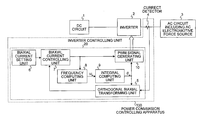•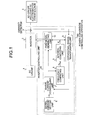•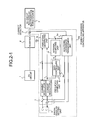•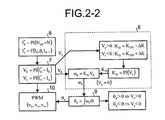•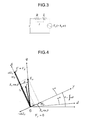•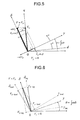•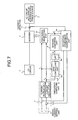•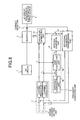•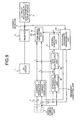••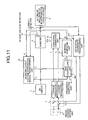•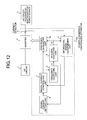•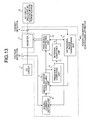•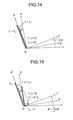•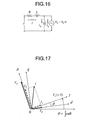•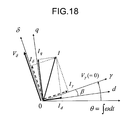•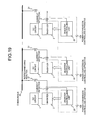•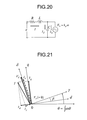••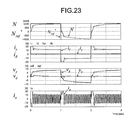•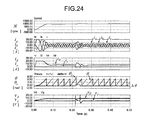•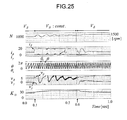•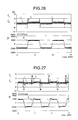•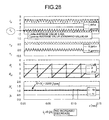•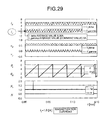•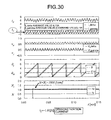•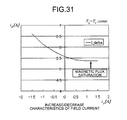•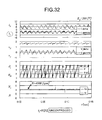•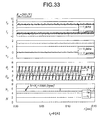•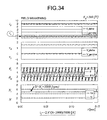•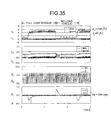•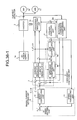•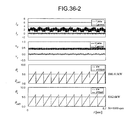•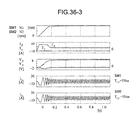•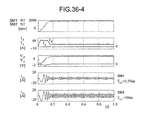•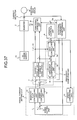•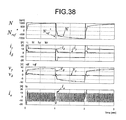•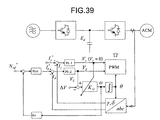•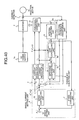••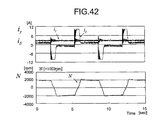•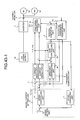••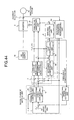•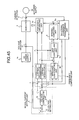•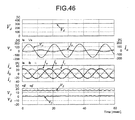•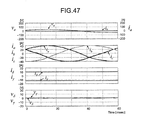•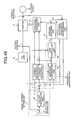••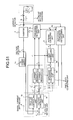•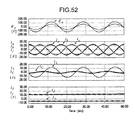## Classifications

• HELECTRICITY
• H02GENERATION; CONVERSION OR DISTRIBUTION OF ELECTRIC POWER
• H02PCONTROL OR REGULATION OF ELECTRIC MOTORS, ELECTRIC GENERATORS OR DYNAMO-ELECTRIC CONVERTERS; CONTROLLING TRANSFORMERS, REACTORS OR CHOKE COILS
• H02P27/00Arrangements or methods for the control of AC motors characterised by the kind of supply voltage
• H02P27/04Arrangements or methods for the control of AC motors characterised by the kind of supply voltage using variable-frequency supply voltage, e.g. inverter or converter supply voltage
• H02P27/06Arrangements or methods for the control of AC motors characterised by the kind of supply voltage using variable-frequency supply voltage, e.g. inverter or converter supply voltage using dc to ac converters or inverters
• HELECTRICITY
• H02GENERATION; CONVERSION OR DISTRIBUTION OF ELECTRIC POWER
• H02MAPPARATUS FOR CONVERSION BETWEEN AC AND AC, BETWEEN AC AND DC, OR BETWEEN DC AND DC, AND FOR USE WITH MAINS OR SIMILAR POWER SUPPLY SYSTEMS; CONVERSION OF DC OR AC INPUT POWER INTO SURGE OUTPUT POWER; CONTROL OR REGULATION THEREOF
• H02M5/00Conversion of ac power input into ac power output, e.g. for change of voltage, for change of frequency, for change of number of phases
• H02M5/40Conversion of ac power input into ac power output, e.g. for change of voltage, for change of frequency, for change of number of phases with intermediate conversion into dc
• H02M5/42Conversion of ac power input into ac power output, e.g. for change of voltage, for change of frequency, for change of number of phases with intermediate conversion into dc by static converters
• H02M5/44Conversion of ac power input into ac power output, e.g. for change of voltage, for change of frequency, for change of number of phases with intermediate conversion into dc by static converters using discharge tubes or semiconductor devices to convert the intermediate dc into ac
• H02M5/453Conversion of ac power input into ac power output, e.g. for change of voltage, for change of frequency, for change of number of phases with intermediate conversion into dc by static converters using discharge tubes or semiconductor devices to convert the intermediate dc into ac using devices of a triode or transistor type requiring continuous application of a control signal
• H02M5/458Conversion of ac power input into ac power output, e.g. for change of voltage, for change of frequency, for change of number of phases with intermediate conversion into dc by static converters using discharge tubes or semiconductor devices to convert the intermediate dc into ac using devices of a triode or transistor type requiring continuous application of a control signal using semiconductor devices only
• H02M5/4585Conversion of ac power input into ac power output, e.g. for change of voltage, for change of frequency, for change of number of phases with intermediate conversion into dc by static converters using discharge tubes or semiconductor devices to convert the intermediate dc into ac using devices of a triode or transistor type requiring continuous application of a control signal using semiconductor devices only having a rectifier with controlled elements
• HELECTRICITY
• H02GENERATION; CONVERSION OR DISTRIBUTION OF ELECTRIC POWER
• H02PCONTROL OR REGULATION OF ELECTRIC MOTORS, ELECTRIC GENERATORS OR DYNAMO-ELECTRIC CONVERTERS; CONTROLLING TRANSFORMERS, REACTORS OR CHOKE COILS
• H02P21/00Arrangements or methods for the control of electric machines by vector control, e.g. by control of field orientation
• H02P21/24Vector control not involving the use of rotor position or rotor speed sensors
• HELECTRICITY
• H02GENERATION; CONVERSION OR DISTRIBUTION OF ELECTRIC POWER
• H02PCONTROL OR REGULATION OF ELECTRIC MOTORS, ELECTRIC GENERATORS OR DYNAMO-ELECTRIC CONVERTERS; CONTROLLING TRANSFORMERS, REACTORS OR CHOKE COILS
• H02P6/00Arrangements for controlling synchronous motors or other dynamo-electric motors using electronic commutation dependent on the rotor position; Electronic commutators therefor
• H02P6/14Electronic commutators
• H02P6/16Circuit arrangements for detecting position
• H02P6/18Circuit arrangements for detecting position without separate position detecting elements

## Abstract

In the present invention, when configuring a control system of an inverter performing power conversion between AC and DC, a frequency computation is performed based on biaxial voltage amounts obtained through two current regulators in such a way that each of biaxial component currents obtained by detecting an AC circuit current and performing a rotational coordinate transformation matches each respective command value and phase information in synchronization with an electromotive force power supply of the AC circuit is obtained by integrating the frequency to perform the rotational coordinate transformation of the AC current and also the inverter is caused to operate by generating a PWM switching signal from the biaxial voltage amounts to perform necessary power conversion control.

## Description

TECHNICAL FIELD
The present invention relates to a power conversion controlling apparatus, a power conversion controlling method, and a power conversion controlling program, and more particularly, relates to a power conversion controlling apparatus, a power conversion controlling method, and a power conversion controlling program that control a power converting unit connected between a DC circuit and an AC circuit including an AC electromotive force source to exchange power between DC and AC with a switching device based on a detection signal of a current flowing through the AC circuit.
BACKGROUND ART
A power conversion controlling apparatus using an inverter is used very widely including application to AC motor generators from the motor control field to the generator control field, application to rectifier circuits and system interconnection inverters that exchange power between an AC power supply and a DC power supply, and the like. These usually have an electromotive force source in an AC circuit and the inverter must be controlled in synchronization with such an electromotive force source.
Thus, to make the inverter work, a switching control signal is generated based on phase information of an AC electromotive force source acquired by means of some sensor or phase information estimated based on the output voltage/current of the inverter and circuit constants of an AC circuit.
A typical electromotive load on the AC circuit side is a synchronous motor and a Hall device, an encoder, a resolver or the like is used as a magnetic pole position sensor to acquire the phase information of the electromotive force in inverter drive particularly of a permanent-magnet synchronous motor or a DC brushless motor. Control modes for detecting such magnetic pole position information can easily deal with high-efficiency operation and high-speed response control, but since a magnetic pole position sensor is needed, problems arise regarding reliability, workability, prices and the like.
On the other hand, various techniques have been proposed to indirectly control the position of rotor from information of the voltage and current of a motor by computation without using such a magnetic pole position sensor. For example, techniques based on detection of induced electromotive force of a motor by rectangular current drive, that based on detection of the voltage when a zero cross point of current in sinusoidal current drive is detected (for example, see Patent Document 1), that based on V/f constant control (for example, see Patent Document 2), and that with a vibration suppression function added to the V/f constant control (for example, see Patent Documents 3 and 4) have been proposed or practically used. However, though the position sensor can be eliminated, conventional control modes have problems that circuit constants of a motor must be incorporated into a control system, such conventional control modes are susceptible to transient changes, the control system will become more complicated, and the like.
Though an induction motor is also considered to be a load having the electromotive force, in comparison with the synchronous motor, the induction motor can be operated without detecting the phase information of the electromotive force and thus, speed control can be performed relatively easily by the V/f constant control and the like. However, the V/f constant control is not E/f constant control and thus, there are problems of lower torque and substantially of responsiveness during low-speed operation. Slip frequency control type vector control or the like is used to ensure fast responsiveness, but with the control system configured by incorporating circuit constants and integrating the induction motor and control device, there are problems that the system configuration will become more complicated and its response characteristics are affected by the circuit constants.
If, on the other hand, an AC generator or a commercial power supply is connected to the AC circuit side and an inverter is used to perform a rectification operation or an interconnected operation from a DC power supply to an AC system, a phase detector of a power supply voltage for generating a control signal in synchronization with the power supply voltage is generally needed. This leads, however, to lower reliability due to a more complicated control system caused by an additional need for an AC power supply voltage detector.
Though a control technique to make a rectification operation or an inversion operation perform by computation with the detected voltage and current of the inverter without using any phase detector of the power supply voltage is known, complicated computation processing is required due to the use of circuit constants (such as the impedance and magnetic flux density of a motor). Also, there is a problem that, for example, it is difficult to deal with wide variations in power supply frequency.
Patent Document 1: Japanese Patent Application Laid-Open No. H5-236789
Patent Document 2: Japanese Patent Application Laid-Open No. 2000-232800
Patent Document 3: Japanese Patent Application Laid-Open No. 2000-236694
Patent Document 4: Japanese Patent Application Laid-Open No. 2000-204694
DISCLOSURE OF INVENTION Problem to be Solved by the Invention
The present invention has been devised in view of the above problems and an object of the present invention is to provide a power conversion controlling apparatus, a power conversion controlling method, and a power conversion controlling program that can perform control of a power converting unit such as an inverter easily with high precision and can be used in a wide range of fields.
Means for Solving Problem
To solve the above problems and to achieve the above objects, a power conversion controlling apparatus according to one aspect of the present invention, controls a power converting unit connected between a DC circuit and an AC circuit including an AC electromotive force source to exchange power between DC and AC with a switching device based on a detection signal detected by a current detector of a current flowing through the AC circuit. The power conversion controlling apparatus includes a frequency computing unit that determines an operating frequency of the power converting unit to output an operating frequency signal, an integral computing unit that computes a phase angle signal by integration from the output of the frequency computing unit to output the phase angle signal, an orthogonal biaxial transforming unit that computes a biaxial current of an active component and a reactive component by orthogonal biaxial transformation based on the detection signal of the current detector and the phase angle signal of the integral computing unit to output the biaxial current, a biaxial current setting unit that determines a command value of the biaxial current to output the command value, a biaxial current controlling unit that computes an amount of error from a difference between the output of the orthogonal biaxial transforming unit and that of the biaxial current setting unit to output an amplitude command value according to the amount of error for each biaxial component, and a PWM signal generating unit that generates a PWM signal controlling the power converting unit based on the output of the biaxial current controlling unit and the phase angle signal of the integral computing unit, wherein the frequency computing unit determines the operating frequency of the power converting unit so as to lead the amplitude command value corresponding to a reactive component of current among the amplitude command values output by the biaxial current controlling unit to zero.
According to another aspect of the present invention, in the power conversion controlling apparatus, the frequency computing unit selects, among the amplitude command values output by the biaxial current controlling unit, a value obtained by multiplying the amplitude command value corresponding to the active component of current by a gain, a value obtained by multiplying a time variation reduced value of the amplitude command value corresponding to the active component of current by the gain, or a time variation reduced value of the gain multiplied value, as the operating frequency of the power converting unit.
According to still another aspect of the present invention, in the power conversion controlling apparatus, the frequency computing unit includes an impedance compensating unit that outputs a compensation value for compensating for a stationary or transient voltage drop in a line impedance portion when the amplitude command value corresponding to the active component of current among the amplitude command values output by the biaxial current controlling unit changes, wherein a value obtained by multiplying an added value of the amplitude command value corresponding to the active component of current or a time variation reduced value of the amplitude command value and an output of the impedance compensating unit by a gain, or a time variation reduced value of the gain multiplied value is selected as the operating frequency of the power converting unit.
According to still another aspect of the present invention, in the power conversion controlling apparatus, the impedance compensating unit computes the compensation value based on the active component of current or a command value of the active component of current.
According to still another aspect of the present invention, in the power conversion controlling apparatus, the impedance compensating unit computes the compensation value by reducing time variations of the active component of current or the command value of the active component of current.
According to still another aspect of the present invention, in the power conversion controlling apparatus, the impedance compensating unit computes the compensation value using, among the amplitude command values output by the biaxial current controlling unit, the amplitude command value corresponding to the reactive component of current.
According to still another aspect of the present invention, in the power conversion controlling apparatus, the frequency computing unit selects a value obtained by multiplying a constant by a gain as the operating frequency of the power converting unit.
According to still another aspect of the present invention, the power conversion controlling apparatus includes an output voltage orthogonal biaxial transforming unit that computes a biaxial voltage by orthogonal biaxial transformation from an output voltage of the power converting unit and the phase angle signal of the integral computing unit to output a signal corresponding to the amplitude command value output by the biaxial current controlling unit for each biaxial component, wherein the frequency computing unit substitutes the amplitude command value with the signal value of the output voltage orthogonal biaxial transforming unit for each biaxial component.
According to still another aspect of the present invention, in the power conversion controlling apparatus, the biaxial current setting unit computes the command value of the active component of current using the operating frequency of the power converting unit output by the frequency computing unit.
According to still another aspect of the present invention, in the power conversion controlling apparatus, the biaxial current setting unit computes the command value of the active component of current using a DC side voltage value of the power converting unit.
According to still another aspect of the present invention, in the power conversion controlling apparatus, a power factor is arbitrarily set by adjusting the command value of the reactive component of current of the biaxial current setting unit.
According to still another aspect of the present invention, in the power conversion controlling apparatus, the biaxial current setting unit determines the command value of the reactive component of current as a value such that the power factor at an output end of the power converting unit becomes 1.
According to still another aspect of the present invention, in the power conversion controlling apparatus, the biaxial current setting unit determines the command value of the reactive component of current as a value such that the power factor at an AC electromotive force source end of the AC circuit becomes 1.
According to still another aspect of the present invention, in the power conversion controlling apparatus, the AC circuit is a circuit including one or a plurality of AC machines.
According to still another aspect of the present invention, in the power conversion controlling apparatus, the AC machine is a synchronous machine, a reluctance machine, an induction machine, or an induction synchronous machine.
According to still another aspect of the present invention, in the power conversion controlling apparatus, magnetization or demagnetization of a magnetic field is caused by adjusting the command value of the biaxial current of the biaxial current setting unit.
According to still another aspect of the present invention, in the power conversion controlling apparatus, a commercial power supply, an AC side output of other power converting unit, or an AC load including a capacitor is connected as an AC electromotive force source of the AC circuit.
According to still another aspect of the present invention, in the power conversion controlling apparatus, the DC circuit is a circuit including a capacitor, a DC power supply, or a DC load.
According to still another aspect of the present invention, in the power conversion controlling apparatus, the power converting unit is an inverter that converts DC power into AC power or an AC-DC converter that converts AC power into DC power.
According to still another aspect of the present invention, a computer program product having a computer readable medium including programmed instructions for controlling a power converting unit connected between a DC circuit and an AC circuit including an AC electromotive force source to exchange power between DC and AC with a switching device based on a detection signal detected by a current detector of a current flowing through the AC circuit, wherein the instructions, when executed by a computer, cause the computer to function as a frequency computing unit that determines an operating frequency of the power converting unit so as to lead a amplitude command value corresponding to a reactive component of current among the amplitude command values to zero, an integral computing unit that computes a phase angle signal by integration from the output of the frequency computing unit to output the phase angle signal, an orthogonal biaxial transforming unit that computes a biaxial current of an active component and a reactive component by orthogonal biaxial transformation based on the detection signal of the current detector and the phase angle signal of the integral computing unit to output the biaxial current, a biaxial current setting unit that determines a command value of the biaxial current to output the command value, a biaxial current controlling unit that computes an amount of error from a difference between the output of the orthogonal biaxial transforming unit and that of the biaxial current setting unit to output the amplitude command value according to the amount of error for each biaxial component, and a PWM signal generating unit that generates a PWM signal controlling the power converting unit based on the output of the biaxial current controlling unit and the phase angle signal of the integral computing unit.
According to still another aspect of the present invention, a power conversion controlling method controls a power converting unit connected between a DC circuit and an AC circuit including an AC electromotive force source to exchange power between DC and AC with a switching device based on a detection signal detected by a current detector of a current flowing through the AC circuit. The power conversion controlling method includes a frequency computing step of determining an operating frequency of the power converting unit so as to lead a amplitude command value corresponding to a reactive component of current among the amplitude command values to zero, an integral computing step of computing a phase angle signal by integration from the output at the frequency computing step to output the phase angle signal, an orthogonal biaxial transforming step of computing a biaxial current of an active component and a reactive component by orthogonal biaxial transformation based on the detection signal of the current detector and the phase angle signal at the integral computing step to output the biaxial current, a biaxial current setting step of determining a command value of the biaxial current to output the command value, a biaxial current controlling step of computing an amount of error from a difference between the output at the orthogonal biaxial transforming step and that at the biaxial current setting step to output the amplitude command value according to the amount of error for each biaxial component, and a PWM signal generating step of generating a PWM signal controlling the power converting unit based on the output at the biaxial current controlling step and the phase angle signal at the integral computing step.
Effect of the Invention
According to the present invention, a power conversion controlling apparatus that controls a power converting unit connected between a DC circuit and an AC circuit including an AC electromotive force source to exchange power between DC and AC with a switching device based on a detection signal detected by a current detector of a current flowing through the AC circuit. The power conversion controlling apparatus includes a frequency computing unit that determines an operating frequency of the power converting unit to output an operating frequency signal, an integral computing unit that determines a phase angle signal by integration from the output of the frequency computing unit to output the phase angle signal, an orthogonal biaxial transforming unit that computes a biaxial current of an active component and a reactive component by orthogonal biaxial transformation based on the detection signal of the current detector and the phase angle signal of the integral computing unit to output the biaxial current, a biaxial current setting unit that determines a command value of the biaxial current to output the command value, a biaxial current controlling unit that computes an amount of error from a difference between the output of the orthogonal biaxial transforming unit and that of the biaxial current setting unit to output an amplitude command value according to the amount of error for each biaxial component, and a PWM signal generating unit that generates a PWM signal for controlling the power converting unit based on the output of the biaxial current controlling unit and the phase angle signal of the integral computing unit, wherein the frequency computing unit determines the operating frequency of the power converting unit so as to lead the amplitude command value corresponding to a reactive component of current among the amplitude command values output by the biaxial current controlling unit to zero. Therefore, it makes effects of being able to provide a power conversion controlling apparatus that can detect an AC current without directly detecting the phase of an electromotive force source of an AC circuit by means of a sensor or the like, control the power converting unit without using the circuit constants, control the power converting unit such as an inverter easily with high precision, and be used in a wide range of fields.
BRIEF DESCRIPTION OF DRAWINGS
FIG. 1 is a basic block diagram of a power conversion controlling apparatus of the present invention;
FIG. 2-1 is a block diagram in biaxial representation of the power conversion controlling apparatus of the present invention;
FIG. 2-2 is a control sequence diagram of the power conversion controlling apparatus of the present invention;
FIG. 3 is a diagram of a single-phase basic equivalent circuit of the power conversion controlling apparatus of the present invention;
FIG. 4 is a diagram of relationships (in consideration of circuit resistance) among biaxial voltage vectors Vδ and Vγ, biaxial current vectors Iδ and Iγ, and an AC electromotive force Ea of the power conversion controlling apparatus of the present invention;
FIG. 5 is a diagram of relationships (without consideration of circuit resistance) among the biaxial voltage vectors Vδ and Vγ, the biaxial current vectors Iδ and Iγ, and the AC electromotive force Ea of the power conversion controlling apparatus of the present invention;
FIG. 6 is a diagram of relationships between phase angles and δ-γ axis components in rotational coordinate transformation of the power conversion controlling apparatus of the present invention;
FIG. 7 is a block diagram of the power conversion controlling apparatus of the present invention, in which a frequency computing unit includes LPF;
FIG. 8 is a block diagram of the power conversion controlling apparatus of the present invention in which the frequency computing unit includes an impedance compensating unit;
FIG. 9 is a block diagram of the power conversion controlling apparatus of the present invention in which Iδ is input into the impedance compensating unit;
FIG. 10 is a block diagram of the power conversion controlling apparatus of the present invention in which Vγ is input into the impedance compensating unit;
FIG. 11 is a block diagram of the power conversion controlling apparatus of the present invention in which an inverter output voltage orthogonal biaxial transforming unit is provided in;
FIG. 12 is a block diagram of the power conversion controlling apparatus of the present invention in which an operating frequency of the inverter is input into a biaxial current setting unit;
FIG. 13 is a block diagram of the power conversion controlling apparatus of the present invention in which a DC voltage of the inverter is input into the biaxial current setting unit;
FIG. 14 is diagram of relationships between voltage and current vectors during power factor 1 operation at an output end of the inverter of the power conversion controlling apparatus of the present invention;
FIG. 15 is diagram of relationships between voltage and current vectors during power factor 1 operation at an electromotive force end of an AC circuit of the power conversion controlling apparatus of the present invention;
FIG. 16 is a diagram of a single-phase equivalent circuit when an electromotive force source involving an excitation circuit is connected to the AC circuit;
FIG. 17 is a diagram of voltage and current vectors when the electromotive force source involving the excitation circuit is connected to the AC circuit;
FIG. 18 is a diagram of voltage and current vectors during power factor 1 operation at the electromotive force end when the electromotive force source involving the excitation circuit is connected to the AC circuit;
FIG. 19 is a diagram of the power conversion controlling apparatuses in which a plurality of inverters are connected;
FIG. 20 is a diagram of a single-phase equivalent circuit when an AC load including a capacitor is connected to the AC circuit;
FIG. 21 is a diagram of voltage and current vectors during power factor 1 operation at a capacitor end when the AC load including the capacitor is connected to the AC circuit;
FIG. 22 is a block diagram of Example 1 when a synchronous motor is connected to the AC circuit in the power conversion controlling apparatus of the present invention;
FIG. 23 is a diagram of simulation analysis results for the block diagram of Example 1 when the synchronous motor is connected to the AC circuit in the power conversion controlling apparatus of the present invention;
FIG. 24 is a diagram of simulation analysis results of transient phase follow-up control for the block diagram of Example 1 when the synchronous motor is connected to the AC circuit in the power conversion controlling apparatus of the present invention;
FIG. 25 is a diagram of simulation analysis results of phase follow-up control by Vδ for the block diagram of Example 1 when the synchronous motor is connected to the AC circuit in the power conversion controlling apparatus of the present invention;
FIG. 26 is a diagram of experimental results of Example 1 when the synchronous motor is connected to the AC circuit in the power conversion controlling apparatus of the present invention;
FIG. 27 is a diagram of experimental results of Example 1 when the synchronous motor is connected to the AC circuit in the power conversion controlling apparatus of the present invention;
FIG. 28 is a diagram of experimental results at Iγ*=0 A in Example 1 when the synchronous motor is connected to the AC circuit in the power conversion controlling apparatus of the present invention;
FIG. 29 is a diagram of experimental results at Iγ*=1.0 A in Example 1 when the synchronous motor is connected to the AC circuit in the power conversion controlling apparatus of the present invention;
FIG. 30 is a diagram of experimental results at Iγ*=−1.0 A in Example 1 when the synchronous motor is connected to the AC circuit in the power conversion controlling apparatus of the present invention;
FIG. 31 is a diagram of experimental characteristics of Example 1 when the synchronous motor is connected to the AC circuit in the power conversion controlling apparatus of the present invention;
FIG. 32 is a diagram of experimental results at Iγ*=0 A and rated DC voltage in Example 1 when the synchronous motor is connected to the AC circuit in the power conversion controlling apparatus of the present invention;
FIG. 33 is a diagram of experimental results at Iγ*=0 A and the raised DC voltage in Example 1 when the synchronous motor is connected to the AC circuit in the power conversion controlling apparatus of the present invention;
FIG. 34 is a diagram of experimental results when demagnetization operation is performed at the rated DC voltage in Example 1 when the synchronous motor is connected to the AC circuit in the power conversion controlling apparatus of the present invention;
FIG. 35 is a diagram of experimental results when a load torque is abruptly changed between total load and no load in Example 1 when the synchronous motor is connected to the AC circuit in the power conversion controlling apparatus of the present invention;
FIG. 36-1 is a diagram of a power conversion system in Example 1 when a plurality of synchronous motors are driven;
FIG. 36-2 is a diagram of operation waveforms when synchronous motors have different ratings in the power conversion system shown in FIG. 36-1;
FIG. 36-3 is a diagram of simulation analysis results when synchronous motors have the same load torque in the power conversion system shown in FIG. 36-1;
FIG. 36-4 is a diagram of simulation analysis results when synchronous motors have different load torques in the power conversion system shown in FIG. 36-1;
FIG. 37 is a block diagram of Example 2 when the synchronous motor is connected to an AC circuit in a power conversion controlling apparatus of the present invention;
FIG. 38 is a diagram of simulation analysis results for the block diagram of Example 2 when the synchronous motor is connected to the AC circuit in the power conversion controlling apparatus of the present invention;
FIG. 39 is a block diagram of Example 3 when the synchronous motor is connected to an AC circuit in a power conversion controlling apparatus of the present invention;
FIG. 40 is a block diagram of Example 4 when the induction motor is connected to an AC circuit in a power conversion controlling apparatus of the present invention;
FIG. 41 is a diagram of simulation analysis results for the block diagram of Example 4 when the induction motor is connected to the AC circuit in the power conversion controlling apparatus of the present invention;
FIG. 42 is a diagram of experimental results of Example 4 when the induction motor is connected to the AC circuit in the power conversion controlling apparatus of the present invention;
FIG. 43-1 is a diagram of a power conversion system when a plurality of induction motors are driven in the system in Example 4;
FIG. 43-2 is a diagram of simulation analysis results when induction motors have the same load torque in the power conversion system shown in FIG. 43-1;
FIG. 43-3 is a diagram of simulation analysis results when induction motors have different load torques in the power conversion system shown in FIG. 43-1;
FIG. 44 is a block diagram of Example 5 when the induction motor is connected to an AC circuit in a power conversion controlling apparatus of the present invention;
FIG. 45 is a block diagram of Example 6 when the AC power supply is connected to an AC circuit and the DC power supply is connected to a DC circuit in a power conversion controlling apparatus of the present invention;
FIG. 46 is a diagram of simulation analysis results for the block diagram of Example 6 when the AC power supply is connected to the AC circuit and the DC power supply is connected to the DC circuit in the power conversion controlling apparatus of the present invention;
FIG. 47 is a diagram of simulation analysis results when the AC power supply is cut off in the block diagram of Example 6 when the AC power supply is connected to the AC circuit and the DC power supply is connected to the DC circuit in the power conversion controlling apparatus of the present invention;
FIG. 48 is a block diagram of Example 7 when the AC power supply is connected to an AC circuit and the DC power supply is connected to a DC circuit in a power conversion controlling apparatus of the present invention;
FIG. 49 is a block diagram of Example 8 when the AC power supply is connected to an AC circuit and the DC power supply is connected to a DC circuit in a power conversion controlling apparatus of the present invention;
FIG. 50 is a diagram of experimental results of Example 7 when the AC power supply is connected to the AC circuit and a resistor is connected to the DC circuit as a DC load in the power conversion controlling apparatus of the present invention;
FIG. 51 is a block diagram of Example 9 when an AC load including a capacitor is connected to an AC circuit in a power conversion controlling apparatus of the present invention; and
FIG. 52 is a diagram of simulation analysis results for the block diagram of Example 9 when the AC load including the capacitor is connected to the AC circuit in the power conversion controlling apparatus of the present invention.
EXPLANATIONS OF LETTERS OR NUMERALS
• 1 DC circuit
• 1 a DC power supply
• 2 inverter
• 3 AC circuit (including an AC electromotive force source)
• 3 b synchronous motor
• 3 c induction motor
• 3 d AC power supply
• 3 e AC circuit (including a capacitor)
• 4 current detector
• 5 orthogonal biaxial transforming unit
• 5 a rotational coordinate transforming unit
• 6 biaxial current setting unit
• 7 biaxial current controlling unit
• 8 frequency computing unit
• 8 a gain controlling unit
• 8 b impedance compensating unit
• 8 c LPF (low-pass filter)
• 9 integral computing unit
• 10 PWM signal generating unit
• 11 speed converting unit
• 12 speed controlling unit
• 13 speed setting unit
• 14 magnetic flux controlling unit
• 15 voltage detector
• 17 DC voltage setting unit
• 18 reactive current controlling Unit
• 20 inverter controlling unit
• 21 AC side voltage detector
• 22 inverter output voltage orthogonal biaxial transforming unit
• 30 common bus
• 31 interconnecting reactor
• 100 power conversion controlling apparatus
BEST MODES FOR CARRYING OUT THE INVENTION
Exemplary embodiments of a power conversion controlling apparatus, a power conversion controlling method, and a power conversion controlling program according to the present invention will be described below with reference to attached drawings. However, the present invention is not limited by such embodiments. Components in the embodiments shown below include those that can easily be conceived by a person skilled in the art or are substantially identical. Meanwhile, it is assumed herein that a power converting unit that exchanges power between DC and AC includes an inverter that converts DC power into AC power and an AC-DC converter that converts AC power into DC power. Though inverters are mainly exemplified as the power converting unit in embodiments that follow, the present invention is also applicable to AC-DC converters.
First Embodiment
FIG. 1 is a diagram of a basic configuration of a power conversion controlling apparatus according to the present invention. The power conversion controlling apparatus according to a first embodiment is used to control an inverter that performs power conversion between DC and AC when an AC circuit has an electromotive force source.
In FIG. 1, numeral 1 is a DC circuit, numeral 2 is an inverter connected between the DC circuit 1 and an AC circuit 3 to exchange power between DC and AC with a switching device, numeral 3 is the AC circuit including the electromotive force source (hereinafter also called an “AC circuit”), and numeral 100 is a power conversion controlling apparatus. In embodiments that follow, numeral 2 is illustrated as an inverter, but numeral 2 may be an AC-DC converter that performs AC-DC conversion depending on types of the connected DC circuit 1 and the AC circuit 3.
The DC circuit 1 is a circuit including a DC power supply, a DC load, or a capacitor. The AC circuit 3 including an AC electromotive force source is an AC circuit having an electromotive force source such as an AC motor, an AC generator, an AC power supply, and a load including a capacitor.
The power conversion controlling apparatus 100 includes a current detector 4 that detects a current flowing through the AC circuit 3 to output a detection signal and an inverter controlling unit 20 that controls the inverter 2 based on the detection signal of the current detector 4.
The inverter controlling unit 20 includes an orthogonal biaxial transforming unit 5, a biaxial current setting unit 6, a biaxial current controlling unit 7, a frequency computing unit 8, an integral computing unit 9, and a PWM signal generating unit 10. The inverter controlling unit 20 may be configured by a microcomputer, DSP or the like and a power conversion controlling program may be executed by a computer to implement functions of the orthogonal biaxial transforming unit 5, biaxial current setting unit 6, biaxial current controlling unit 7, frequency computing unit 8, integral computing unit 9, and PWM signal generating unit 10.
The frequency computing unit 8 determines an operating frequency of the inverter 2 to output an operating frequency signal ωe. In this case, the frequency computing unit 8 determines the operating frequency signal ωe of the inverter 2 in such a way that the amplitude command value corresponding to a reactive component of current among the amplitude command values output by the biaxial current controlling unit 7 is led to zero. Details of this principle will be described later.
The integral computing unit 9 computes a phase angle signal θe by integration from the output of the frequency computing unit 8 and outputs the phase angle signal θe. The orthogonal biaxial transforming unit 5 computes a biaxial current of an active component and a reactive component by orthogonal biaxial transformation from the detection signal of the current detector 4 and the phase angle signal θe of the frequency computing unit 8 to output the biaxial current. The biaxial current setting unit 6 determines a command value of the biaxial current to output the command value. The biaxial current controlling unit 7 computes an amount of error from a difference between the output of the orthogonal biaxial transforming unit 5 and that of the biaxial current setting unit 6 to output an amplitude command value according to the amount of error for each biaxial component. The PWM signal generating unit 10 generates a PWM signal, which is a control signal to be provided to the inverter 2, based on the output of the biaxial current controlling unit 7 and the phase angle signal θe of the integral computing unit 9 to supply the PWM signal to the inverter 2.
FIG. 2-1 is a diagram of the configuration of the power conversion controlling apparatus that performs a frequency computation using an amplitude command value (an inverter voltage command value in a broad sense) in the power conversion controlling apparatus in FIG. 1. In FIG. 2-1, the same numerals are attached to components having functions equivalent to those of components in FIG. 1 to omit a description of common portions. The power conversion controlling apparatus 100 in FIG. 2-1 depicts a concrete configuration example when a three-phase (UVW) AC load including an AC electromotive force source is connected to the AC circuit 3 in the power conversion controlling apparatus in FIG. 1. The inverter 2 is a three-phase inverter and can be configured by a bridge circuit including a switching device such as an IGBT.
In FIG. 2-1, the current detector 4 detects at least two phases of current flowing through the AC circuit 3 as a detection signal. A rotational coordinate transforming unit 5 a, which is the orthogonal biaxial transforming unit, performs a δ-γ transformation of a detection signal detected by the current detector 4 in which the active component is set to a γ-axis component and the reactive component is set to a γ-axis component in synchronization with the operating frequency of the inverter 2 to output transformed biaxial amounts Iγ and Iδ to the biaxial current controlling unit 7. The biaxial current controlling unit 7 generates biaxial control voltages of the inverter 2, that is, amplitude command values Vγ and Vδ of the inverter 2 via two sets of current regulators 1 and 2 included in the biaxial current controlling unit 7 in such a way that the transformed biaxial amounts Iγ and Iδ match biaxial current command values Iγ* and Iδ* output by the biaxial current setting unit 6.
The frequency computing unit 8 (gain controlling unit 8 a) determines the operating frequency signal ωe of the inverter 2 in such a way that the uniaxial voltage value Vγ of the amplitude command values Vγ and Vδ becomes zero, and the integral computing unit 9 obtains the operating phase angle signal θe of the inverter 2 by integrating the operating frequency signal ωe to perform the rotational coordinate transformation of the AC current and also causes the PWM signal generating unit 10 to generate a PWM signal (vu, vv, vw), which is a control signal of the inverter 2, by trigonometry or the like from biaxial voltage amounts Vγ and Vδ to operate the inverter 2. Accordingly, it becomes possible to control power exchange between AC and DC only by detection control of current flowing through the AC circuit without acquiring phase information of an AC electromotive force source of the AC circuit 3 by a sensor and using circuit constants.
Next, the control principle of the inverter in the power conversion controlling apparatus of the above configuration will be described in detail. FIG. 2-2 is a control sequence diagram of the power conversion controlling apparatus in FIG. 2-1. FIG. 3 is a diagram of a single-phase equivalent circuit when a synchronous motor and an AC power supply are connected as an example that an electromotive force source in the AC circuit 3 is included. In FIG. 3, R denotes a circuit resistor, L denotes a circuit inductance, and Ea denotes a transformation amount of rotational coordinates of an AC electromotive force source ea. Here, the AC electromotive force source is proportional to the angular frequency ω when a motor is connected, but the voltage takes an approximately constant value when a commercial AC power supply is connected. Rotational coordinate transformation under the formula (1) based on a circuit equation of the equivalent circuit of FIG. 3 yields the formula (2) shown below.
[Formula 1]
θ=∫ωdt  (1)
$[ Formula ⁢ ⁢ ⁢ 2 ]$ $V γ = R ⁢ I γ + L ⁢ ⅆ I γ ⅆ t - ω ⁢ ⁢ LI δ + E a ⁢ sin ⁢ ⁢ β ⁢ ⁢ V δ . = RI δ + L ⁢ ⅆ I δ ⅆ t + ω ⁢ ⁢ LI γ + E a ⁢ cos ⁢ ⁢ β ( 2 )$
Here, Vδ is a voltage command value component proportional to an output voltage vector of the inverter 2, Vγ is a voltage command value component orthogonal to this axis, Iδ is the active component of current of a current vector, and Iγ is the reactive component of current. β is an angle of phase difference between the output voltage vector Vδ of the inverter 2 and a voltage vector Ea of AC electromotive force. Since differential terms are zero in a stationary state in the formula (2), the formula (3) shown below is obtained,
[Formula 3]
V γ =RI γ −ωLI δ +E a sin β
V δ =RI δ +ωLI γ +E a cos β  (3)
FIG. 4 depicts a vector diagram when the component voltage Vγ of the γ axis is zero in a stationary state with the biaxial component voltages Vδ and Vγ, biaxial component currents Iδ and Iγ, and AC electromotive force Ea based on the formula (3). Here, it is assumed that coordinate axes of the biaxial component voltages Vδ and Vγ are the δ axis and γ axis, the voltage vector Ea of the AC electromotive force is the q axis, and a magnetic flux axis orthogonal to the q axis is the d axis. Here, β denotes a phase angle between two coordinate axes. FIG. 5 depicts a vector diagram when a voltage drop by circuit resistance is ignored. Now, if controlled to be Iγ=0 and Iδ=const., the formula (3) is further simplified to the formula (4) shown below.
[Formula 4]
V γ =−ωLI γ +E a sin β
V δ =RI δ +E a cos β  (4)
Here, if controlled to be Vγ=0, the formula (5) shown below can be obtained from the formula (4).
$[ Formula ⁢ ⁢ 5 ] I δ = E a ω ⁢ ⁢ L ⁢ sin ⁢ ⁢ β ( 5 )$
At this time, power Pa from the inverter 2 is given by the formula (6) shown below, which is represented by a sine-wave function of an angle of phase difference or load angle β.
$[ Formula ⁢ ⁢ 6 ] P a = V δ ⁢ I δ = V δ ⁢ E a ω ⁢ ⁢ L ⁢ sin ⁢ ⁢ β ( 6 )$
If the angle of phase difference (or load angle) β is small, the formula (4) can be approximated by the formula (7) shown below.
[Formula 7]
V γ ≈−ωLI δ +E aβ
V δ ≈RI δ +E a  (7)
From the VS formula of the formula (7), the AC electromotive force source Ea is obtained from the formula (8) shown below.
[Formula 8]
E a ≈V δ −RI δ  (8)
Further, if a voltage drop RIδ caused by circuit resistance can be ignored, the formula (8) will be the formula (9) shown below.
[Formula 9]
Ea≈Vδ  (9)
At this time, the AC electromotive force Ea is approximated from the output voltage vector value Vδ. If the AC electromotive force source derives from an AC motor, the AC electromotive force source is proportional to the angular frequency ω and given by the formula (10) shown below.
[Formula 10]
Ea=kΨω  (10)
Here, kΨ is a proportionality constant proportional to the magnetic flux. If Vγ=0, from the formulas (7) and (10), the angle of phase difference β can be approximated by the formula (11) shown below.
[Formula 11]
β≈(L/k Ψ)I δ  (11)
Further, from the formulas (9) and (10), the angular frequency ω can be approximated by the formula (12) shown below and is proportional to Vδ.
[Formula 12]
ω≈V δ /k Ψ  (12)
From the formula (12), if an appropriate proportional gain for Vδ is KG, the angular frequency ω of the AC electromotive force source can be considered basically to be ω shown below
[Formula 13]
ωe=KGVδ  (13)
The integral computing unit 9 can calculate a rotational phase angle θe of the inverter 2 in synchronization with the rotational phase angle of the AC electromotive force source according to the formula (14) shown below with the operating frequency ωe of the inverter of the formula (13) as an input.
[Formula 14]
θe=∫ωedt  (14)
Here, if the rotational phase angle θe obtained from the frequency computation does not match an appropriate phase angle θ synchronized with the rotation phase angle of the AC electromotive force source, the γ-axis component voltage Vγ of the output voltage vector V of the inverter 2 shown in FIG. 6 appears. This Vγ takes a negative value when the phase angle θe obtained from the computation lags and a positive value when the phase angle leads. Thus, the rotational phase angle θe obtained from the computation can be led to the appropriate rotational phase angle θ by adjusting the value of KG in the formula (13) so that the value of Vγ becomes zero through a PI controller or the like based on the sign of the γ-axis component voltage Vγ.
The power conversion controlling apparatus according to the first embodiment includes the frequency computing unit 8 that determines an operating frequency of the inverter 2 to output the operating frequency signal ωe, the integral computing unit 9 that determines a phase angle signal θe by integration from the output of the frequency computing unit 8 to output the phase angle signal, the orthogonal biaxial transforming unit 5 that computes a biaxial current of the active component and reactive component by orthogonal biaxial transformation from a detection signal of the current detector 4 and the phase angle signal θe of the frequency computing unit 8 to output the biaxial current, the biaxial current setting unit 6 that determines a command value of the biaxial current to output the command value of the biaxial current, the biaxial current controlling unit 7 that computes an amount of error from a difference between output of the orthogonal biaxial transforming unit 5 and that of the biaxial current setting unit 6 to output an amplitude command value according to the amount of error for each biaxial component, and the PWM signal generating unit 10 that generates a control signal to be provided to the inverter 2 from output of the biaxial current controlling unit 7 and the phase angle signal θe of the integral computing unit 9, and the frequency computing unit 8 determines the operating frequency ωe of the inverter 2 in such a way that the amplitude command value corresponding to the reactive component of current among the amplitude command values output by the biaxial current controlling unit 7 is led to zero. Therefore, it becomes possible to control the inverter only by detection of an AC current without detecting the phase of an electromotive force source of the AC circuit by a phase detection sensor and using circuit constants when controlling the inverter to enable desired power conversion between AC and DC, to control the inverter easily with high precision, and to use the inverter in a wade range of fields. To add a remark, the power conversion controlling apparatus according to the first embodiment can be used for general purposes because no AC circuit constant is used.
Meanwhile, the frequency computing unit 8 may use a value obtained by multiplying the constant by a gain as the operating frequency ωe of the inverter 2. Since the amplitude command value corresponding to the active component of current among the amplitude command values output by the biaxial current controlling unit 7 takes a constant value if the AC electromotive force source has a constant frequency like a commercial power supply, instead of using the amplitude command value as an input into the frequency computing unit 8, the corresponding constant value may be used as an input to be multiplied by a gain in such a way that the amplitude command value corresponding to the reactive component of current among the amplitude command values output by the biaxial current controlling unit 7 becomes zero before being selected as the operating frequency ωe of the inverter 2. The control system can thereby be simplified.
Second Embodiment
A power conversion controlling apparatus according to a second embodiment uses, in the frequency computing unit 8 (gain controlling unit 8 a) of the power conversion controlling apparatus in FIG. 2-1, a value obtained by multiplying the amplitude command value corresponding to the active component of current among the amplitude command values output by the biaxial current controlling unit 7 by a gain, that obtained by multiplying a time variation reduced value of the amplitude command value corresponding to the active component of current by a gain, or a time variation reduced value of such a gain multiplied value is selected as the operating frequency ωe of the inverter 7.
More specifically, the operating frequency ωe of the inverter 2 is determined by multiplying the amplitude command value corresponding to the active component of current by a gain among the amplitude command values output from the biaxial current controlling unit 7. In this case, instead of the amplitude command value corresponding to the active component of current, a time variation reduced value of the amplitude command value corresponding to the active component of current may also be used. Also, instead of using such a gain multiplied value as the operating frequency ωe of the inverter 2, a time variation reduced value of the gain multiplied value may also be determined as the operating frequency of the inverter 2. Time variation reduction at this time is intended to prevent control instability caused by a rapid change of the operating frequency of the AC circuit 3 compared with a case when no such rapid change occurs.
FIG. 7 is a diagram exemplifying the power conversion controlling apparatus when an LPF (low-pass filter) is provided in the frequency computing unit 8 to reduce time variations. In FIG. 7, Vγ and Vδ are output from the biaxial current controlling unit 7 and include some ripple components and thus, if output from the gain controlling unit 8 a is directly used as the operating frequency and phase control, which is an integral thereof, operations may become unstable due to an influence of ripples. In order to reduce the influence of ripple components, output of the gain controlling unit 8 a is used, after being caused to pass through an LPF (low-pass filter) 8 c, as the operating frequency and a control phase signal, which is an integral thereof. The filter factor of the LPF 8 c may be set, for example, as k/(1+τ).
Vδ output by the biaxial current controlling unit 7 may also be caused to pass through the LPF (low-pass filter) before being input into the frequency computing unit 8 to reduce the influence of ripple components.
The power conversion controlling apparatus according to the second embodiment uses, among the amplitude command values output by the biaxial current controlling unit 7, a value obtained by multiplying the amplitude command value corresponding to the active component of current by a gain, that obtained by multiplying a time variation reduced value of the amplitude command value corresponding to the active component of current by a gain, or time variation reduced values of such gain multiplied values is selected as the operating frequency of the inverter 2 and therefore, stable operation can be performed even if the operating frequency of the AC circuit changes rapidly.
Third Embodiment
FIG. 8 is a diagram of an AC power conversion controlling apparatus according to a third embodiment. In FIG. 8, the same numerals are attached to components having functions equivalent to those of components in FIG. 2-1 to omit a description of common portions. The power conversion controlling apparatus according to the third embodiment has a configuration in which an impedance compensating unit is provided in the frequency computing unit 8.
In FIG. 8, the frequency computing unit 8 is provided with an impedance compensating unit 8 b that outputs a compensation value for compensating for a stationary or transient voltage drop in a line impedance portion when an amplitude command value corresponding to the active component of current among the amplitude command values output by the biaxial current controlling unit 7 changes. The frequency computing unit 8 adopts a value obtained by multiplying an added value of an amplitude command value corresponding to the active component of current or a time variation reduced value of the amplitude command value by, for example, an LPF (not shown) and an output of the impedance compensating unit 8 b by a gain or a time variation reduced value of the gain multiplied value by, for example, an LPF as the operating frequency of the inverter 2.
The phase angle θe obtained from the formulas (13) and (14) can easily be made to match the rotational phase angle θ of the AC electromotive force source if the term of Ea in the formula (2) is large. However, as the term of Ea in the formula (2) becomes smaller, it becomes more difficult to perform follow-up control of the phase angle θe obtained by computation to an appropriate phase angle θ. Thus, frequency phase follow-up properties can be improved by removing terms due to an impedance voltage drop shown in the first and second terms from Vδ in the formula (2).
An impedance compensation method will be described below in detail. First, substituting the δ axis voltage Vδ given by the formula (2) into the formula (13) showing a relationship between Vδ and ωe yields the formula (15) shown below.
$[ Formula ⁢ ⁢ 15 ] ω e = K G ⁡ [ RI δ + L ⁢ ⅆ I δ ⅆ t + ω ⁢ ⁢ LI γ + E a ⁢ cos ⁢ ⁢ β ] ( 15 )$
Here, if a voltage drop and a transient current by circuit resistance can be ignored to control Iγ to 0, the formula (16) shown below can be obtained.
[Formula 16]
ωe≈KGEa cos β  (16)
If the AC electromotive force is assumed to be a motor, substituting the formula (10) yields the formula (17) shown below.
[Formula 17]
ωe≈KGkΨω cos β  (17)
From the formula (17), if ωe=ω, the computation proportional gain KG will be a value shown by the formula (18) shown below.
[Formula 18]
K G≈1/(k Ψ cos β)  (18)
The proportional gain KG in the formula (18) will be an approximate value of the appropriate proportional gain KG in the formula (13). If, in the formula (15), Ea cos β is large, the voltage drop RIδ by resistance can be ignored and also the second term dIδ/dt, which is a transient term, can be ignored due to a near stationary state. Therefore, the computed phase angle θe can be made to match the appropriate phase angle θ by tuning the proportional gain KG near the approximate value according to the formula (18) so that the γ-axis component voltage Vγ of the inverter output voltage vector V becomes zero.
If, in the formula (15), Ea cos β is small, an influence of line impedance drop of the first and second terms in the formula (15) grows and the ratio of the fourth term Ea proportional to the frequency falls. Therefore, the appropriate proportional gain KG must change significantly and the frequency phase follow-up properties by the γ-axis component voltage Vδ significantly deteriorates. Thus, frequency phase follow-up properties can be improved by an amount obtained by subtracting a voltage ΔV compensating for a stationary/transient line impedance voltage drop in Vδ of the formula (15) being input into the frequency computing unit. A relationship between ωe and Vδ in this case is shown by the formula (19) shown below.
[Formula 19]
ω=K G(V δ −ΔV)  (19)
Accordingly, the frequency phase follow-up properties of the frequency when the amplitude command value of the active component of current changes can be improved.
The impedance compensating unit 8 b may compute the compensation value using the active component of current or a command value of the active component of current. The configuration of the power conversion controlling apparatus in this case is shown in FIG. 9. More specifically, the resistance drop term and the differential drop term are included in ΔV in the formula (19) to which a compensating term of impedance voltage drop is added to change the form to the formula (20) shown below and the impedance compensating unit 8 b compensates for the impedance voltage drop based on the active component of current Iδ or its setting value Iδ*.
$[ Formula ⁢ ⁢ 20 ] ω e = K G ⁡ [ V δ - ( RI δ + L ⁢ ⅆ I δ ⅆ t ) ] ( 20 )$
The impedance compensating unit 8 b may also compute the compensation value by reducing time variations of the active component of current or the command value of the active component of current. Control instability caused by a rapid change of the operating frequency of the AC circuit 3 compared with a case when no such rapid change occurs is thereby prevented. For example, if the operating frequency ωe of the AC circuit 3 is extremely low, the current value detected by the current detector 4 will be small and the variation width ratio will be high in the detected current value. Thus, the variation ratio will also be high in the active component of current input into the impedance compensating unit 8 b and current variations may excessively affect the compensation in the impedance compensating unit 8 b. Therefore, by adding a filter for reducing time variations to a portion of the impedance compensating unit 8 b where the active component of current is input, the frequency phase follow-up properties can be improved.
Also, the impedance compensating unit 8 b may compute the compensation value using the amplitude command value corresponding to the reactive component of current among the amplitude command values output by the biaxial current controlling unit 7. The configuration of the power conversion controlling apparatus in this case is shown in FIG. 10. Since the amplitude command value Vγ varies corresponding to the reactive component of current particularly during transient change under the influence of impedance voltage drop, the impedance compensating unit 8 b may finally obtain a appropriate operating phase by making adjustments based on the formula (21) shown below using Vγ as ΔV.
[Formula 21]
ωe =K G(V δ −k γ V γ)  (21)
Here, kγ is a proportionality factor for providing an appropriate compensation amount.
Fourth Embodiment
FIG. 11 is a diagram of a power conversion controlling apparatus according to a fourth embodiment. In FIG. 11, the same numerals are attached to components having functions equivalent to those of components in FIG. 2-1 to omit a description of common portions. As shown in FIG. 11, the power conversion controlling apparatus according to the fourth embodiment includes an AC side voltage detector 21 that detects an output voltage of the inverter 2 and an inverter output voltage orthogonal biaxial transforming unit 22 that performs an orthogonal biaxial transformation of an output voltage of the inverter 2. The inverter output voltage orthogonal biaxial transforming unit 22 computes a biaxial voltage by means of orthogonal biaxial transformation from the output voltage of the inverter 2 detected by the AC side voltage detector 21 and the phase angle signal θe of the integral computing unit 9 to output a signal of the amount corresponding to the amplitude command values Vδ and Vγ output by the biaxial current controlling unit 7 for each biaxial component to the frequency computing unit 8. The frequency computing unit 8 computes the operating frequency ωe of the inverter 2 by replacing the amplitude command value with a signal value of the inverter output voltage orthogonal biaxial transforming unit 22 for each biaxial component and using these values.
Fifth Embodiment
FIG. 12 is a diagram of a power conversion controlling apparatus in a fifth embodiment. In FIG. 12, the same numerals are attached to components having functions equivalent to those of components in FIG. 2-1 to omit a description of common portions.
In FIG. 12, the biaxial current setting unit 6 computes a command value of the active component of current using the operating frequency ωe of the inverter 2 output by the frequency computing unit 8. For example, when speed control of an AC machine connected to the AC side is performed, a rotational angular velocity ωme, of the AC machine may be computed by the formula (22) shown below using the operating frequency ωe of the inverter 2.
$[ Formula ⁢ ⁢ 22 ] ω me = 2 p ⁢ ω e ⁢ ⁢ ( SYNCHRONOUS ⁢ ⁢ MOTOR ) ⁢ ⁢ ω me = 2 p ⁢ ω e ⁡ ( 1 - s ) ≈ 2 p ⁢ ω e ⁢ ⁢ ( INDUCTION ⁢ ⁢ MOTOR ) ( 22 )$
Here, p denotes the number of poles of the motor and s denotes a slip of the induction motor. That is, a speed control loop can be formed, without using a speed sensor, by using an output of a speed regulator as the command value of the active component of current via the speed regulator by comparing with a speed setting value of the AC machine based the operating frequency ωe of the inverter 2. Further, the operating frequency ωe of the inverter 2 is not limited to speed settings of the AC machine and can be used also for settings of the reactive component of current in, for example, reactive power compensation.
Sixth Embodiment
FIG. 13 is a diagram of a power conversion controlling apparatus according to a sixth embodiment. In FIG. 13, the same numerals are attached to components having functions equivalent to those of components in FIG. 2-1 to omit a description of common portions. In FIG. 13, the biaxial current setting unit 6 computes the command value of the active component of current using the DC side voltage value of the inverter 2 detected by a voltage detector 15. If power is exchanged between the DC circuit 1 and the AC circuit 3 when the voltage of the DC circuit 1 is not determined, a DC voltage control loop can be formed by detecting the DC voltage, comparing the DC voltage with the DC voltage setting value, and using the output of the voltage regulator as the command value of the active component of current via the voltage regulator. Here, the DC circuit 1 is a DC load accompanied by DC voltage variations caused by a DC current, a DC power supply such as solar cells and fuel cells, or a DC circuit to which only a capacitor is connected such as an active filter and a reactive power compensating unit.
Seventh Embodiment
In a power conversion controlling apparatus in a seventh embodiment, the power factor is set arbitrarily in the power conversion controlling apparatus shown in FIG. 2-1 by adjusting the command value of the reactive component of current of the biaxial current setting unit 6. By regulating the reactive component of current, power factor 1 control at an output end of the inverter 2, power factor 1 control at an AC electromotive force source end of the AC circuit 3, and an operation as a reactive power compensating apparatus can be caused.
The biaxial current setting unit 6 determines the command value of the reactive component of current so that the power factor at the output end of the inverter 2 becomes 1. FIG. 14 is a diagram of relationships between voltage and current vectors during power factor 1 operation at the output end of the inverter of the power conversion controlling apparatus according to the seventh embodiment.
If, as shown in FIG. 14, the rotational phase angle θe of the inverter 2 can be made to match the rotational phase angle θ of the frequency of the AC electromotive force, like the voltage vector Vδ, the current vector becomes a δ-axis component current Iδ only when Iγ is controlled to 0 and Iδ to be constant so that the power factor at an inverter output end can be controlled to be 1. Accordingly, the power conversion controlling apparatus can be operated at high efficiency.
The biaxial current setting unit 6 determines the command value of the reactive component of current so that the power factor at the AC electromotive force source end of the AC circuit 3 becomes 1. By setting the command value in such a way that the power factor at the AC electromotive force end becomes 1, response characteristics of an AC motor can further be enhanced. FIG. 15 is a diagram of relationships between voltage and current vectors during power factor 1 operation at an electromotive force end of the AC circuit 3 of the power conversion controlling apparatus. In FIG. 15, an induced voltage vector Ea of an AC machine matching the q axis and the current vector can be matched by giving the setting value Iγ* of the γ-axis component current Iγ by the formula (23) shown below, and can realize a power factor 1 operation at the electromotive force end. Maximum power can thereby be supplied to the AC electromotive force source and thus, high-speed response characteristics can be given.
[Formula 23]
Iγ=Iδ tan β  (23)
Here, if the AC electromotive force source derives from an AC motor, the angular frequency of the AC electromotive force source is proportional to ω and substituting the formula (11) into the formula (23) when β is small yields the formula (24) shown below.
[Formula 24]
I γ≈(L/k Ψ)I δ 2  (24)
Thus, as shown in the formula (24), the setting value Iγ* of the γ-axis component current Iγ is given as a product of Iδ2, which is an amount of the square of δ-axis component current, and (L/kΨ). Since, as shown in the formula (10), kΨ is a proportionality constant proportional to the magnetic flux, kΨ is known and a constant value and 1/kΨ is also a constant value.
Eighth Embodiment
The AC circuit 3 may be made to be a circuit including one or a plurality of AC machines. If the AC circuit 3 is configured by a circuit including one AC machine, power can be exchanged with the DC circuit 1 by connecting the AC machine as the electromotive force source. If the AC circuit 3 is configured by a circuit including a plurality of AC machines, the plurality of AC machines are connected to one inverter in parallel and the plurality of AC machines are considered virtually to be one AC machine for operation/control.
A synchronous machine, a reluctance machine, an induction machine, or a synchronous induction machine can be used as the AC machine. If a synchronous machine is configured as the AC machine, a wide range of synchronous machines from ones having a field winding including saliency and non-salient poles to permanent-magnet synchronous machines can be applied. If a reluctance machine is configured as the AC machine, reluctance machines including neither field winding nor magnet become applicable by setting the reactive component of current and active component of current appropriately.
FIG. 16 depicts a single-phase equivalent circuit when viewed from a stator side of an induction machine. In FIG. 16, R denotes a wire wound resistor, L a wire wound inductance, and Ea an electromotive force source proportional to the frequency, and ω and Lm shows an exciting inductance.
In the induction machine, a d-axis exciting component current Id=I0 must be flown in addition to a q-axis torque component current Iq when the induced voltage vector is made to match the q axis. FIG. 17 is a diagram of voltage and current vectors when the electromotive force source involving an excitation circuit is connected to the AC circuit. In FIG. 17, the output voltage vector of the inverter 2 is assumed to have only a δ-axis component and voltage and current vectors when a current vector obtained by adding a d-axis exciting component current Id to a δ-axis component current Iδ is I are shown. In this case, a γ-axis component current Iγ is given by the formula (25) shown below.
[Formula 25]
Iγ=I0 cos β  (25)
Here, FIG. 18 depicts voltage and current vectors when the current of the induction machine is controlled by a resultant current vector I from the q-axis torque component current Iq and the d-axis exciting component current Id=I0. In this case, the current vector I can be decomposed into the δ-axis component current Iδ and the γ-axis component current Iγ, forming the exemplified relationships. The γ-axis component current Iγ is given by the formula (26) shown below.
[Formula 26]
I γ =I 0 cos β+I δ tan β  (26)
Here, substituting the formula (24) assuming that β is small yields the formula (27) shown below.
[Formula 27]
I γ ≈I 0+(L/k Ψ)I δ 2  (27)
Since vector control of the biaxial component current can independently be performed, response characteristics can be enhanced when compared with the general V/f constant control. By providing the setting value Iγ* of the reactive component of current by the formula (27), the power factor between the torque component current Iq and the AC electromotive force Ea excluding the exciting component current Id becomes 1, enabling still faster control.
Since magnetization can be caused by flowing a lagging reactive component of current Iγ of the biaxial component current in the power conversion controlling apparatus, a high torque can be caused without increasing the active component of current Iδ significantly. Also, demagnetization can be caused by flowing a leading reactive component of current Iγ, enabling high-speed operation control without raising the voltage.
Though increasing/decreasing the lagging reactive component of current Iγ is affected by a saturation phenomenon of the magnetic circuit, the power conversion controlling apparatus can be controlled without being affected by magnetization/demagnetization control by the reactive component of current Iγ because sensorless control of the present invention does not depend on any estimation method using a motor model even though the magnetic flux model of the motor is unknown. By adjusting the command value of the biaxial current of the biaxial current setting unit 6, as described above, magnetization or demagnetization of a magnetic field can be caused.
Ninth Embodiment
As the AC electromotive force source of the AC circuit 3, for example, a commercial power supply, an AC side output of another inverter, or an AC load including a capacitor may be used. The AC side output of another inverter may be connected via a serially coupled reactor as the AC circuit 3 to mutually exchange power. FIG. 19 is a diagram of the power conversion controlling apparatuses in which a plurality of inverters are connected. FIG. 19 can suitably be used when only weak electric power systems are interconnected. Loads are connected to a common bus 30 and the inverter 2 is connected to the common bus 30 via an interconnecting reactor 31.
A case when an AC circuit including a capacitor via a serially coupled reactor is connected as the AC circuit 3 will be described. In this case, the voltage charged in the capacitor acts as the AC electromotive force source of the AC circuit 3. FIG. 20 is a single-phase equivalent circuit, and R denotes a wire wound resistor, L a wire wound inductance, C a capacitor, and Ea a load connected to the capacitor in parallel. FIG. 21 depicts a relationship diagram of voltage and current vectors with respect to the δ-γ axis and the q-d axis when a current in phase with the capacitor terminal voltage flows through the load. In addition to the active component of current Iq to the load, the leading reactive component of current Id must be flown and the resultant current vector I of such current vectors can be decomposed into the δ-axis component current Iδ and the γ-axis component current Iγ, forming the exemplified relationships.
In the power conversion controlling apparatus according to the ninth embodiment, power exchange between DC and AC, that is, power conversion when the AC circuit that can exchange active power between the AC electromotive force source and inverter output can be performed. An AC power supply failure state in which some failure occurs in a commercial power supply or a generator constituting the AC power supply can also be detected by the power conversion controlling apparatus.
Tenth Embodiment
The DC circuit 1 of the power conversion controlling apparatus may be, for example, a circuit including a capacitor, a DC power supply, or a DC load. If the DC circuit 1 is configured with the circuit including a capacitor, reactive power can be exchanged between the DC circuit side and AC circuit side even in a state in which only a capacitor is connected on the DC circuit side and therefore, the apparatus can be operated as a reactive power compensating apparatus or an active filter.
If the DC circuit 1 is configured with the circuit including the DC power supply, this means that power is exchanged from the DC power supply to the AC power supply. If, for example, an AC motor is connected to the AC circuit 3, the DC power supply can be used as a drive energy source of an inverter and, if an AC generator or a commercial power supply is connected to the AC circuit 3, the DC power supply can be used as an energy source to transfer power to the AC circuit side. Also, when charging, for example, an accumulator in the DC circuit from the AC circuit side, the DC power supply can be used as an energy source to transfer power to the AC circuit side. If the DC circuit 1 is configured with the circuit including a DC load, power can be supplied from a generator or AC power supply of the AC circuit to the DC load in the DC circuit.
Meanwhile, the present invention is not limited to the above first to tenth embodiments and can be carried out by combining each of the first to tenth embodiments. The above embodiments have been described using a three-phase AC, but the present invention is not limited to this and is applicable to any two-phase AC or higher. Examples 1 to 9 of power conversion control systems to which the power conversion controlling apparatuses according to the first to tenth embodiments are applied will be described below.
Example 1
FIG. 22 is a diagram of a power conversion control system according to Example 1. The power conversion control system shown in FIG. 22 performs speed control of a synchronous motor 3 b without using a rotational position sensor or a speed sensor by connecting the synchronous motor 3 b as an AC machine including an electromotive force source to the AC circuit 3 from a DC power supply 1 a, which is the DC circuit 1, via the inverter 2.
A current flowing through the synchronous motor 3 b is detected by the current detector 4. A coordinate transformation is performed by the rotational coordinate transforming unit 5 a, which is the orthogonal biaxial transforming unit 5, and voltage command values (that is, amplitude command values) Vδ and Vγ are generated via the biaxial current controlling unit 7 so that rotational coordinate-transformed biaxial amounts Iδ and Iγ match corresponding biaxial current setting values Iδ* and Iγ* respectively to output the voltage command values to the PWM signal generating unit 10 and the frequency computing unit 8.
The PWM signal generating unit 10 generates a PWM signal to control the inverter 2. The amplitude command values Vδ and Vγ are at the same time used by the gain controlling unit 8 a of the frequency computing unit 8 to determine the operating frequency ωe of the inverter by multiplying the amplitude command value Vδ by a gain so that Vγ becomes zero. By integrating the operating frequency of the inverter by the integral computing unit 9, the phase angle signal θe made to match the phase angle θ of the internal electromotive force of the synchronous motor 3 b is obtained.
Meanwhile, the impedance compensating unit 8 b is effective for compensating for being incapable of controlling Vγ to zero when the reference value Iδ* of the active component of current changes markedly due, for example, to acceleration or deceleration. The case is shown here in which a compensation is made based on the active component of current command value Iδ*. The active component of current command value Iδ* is obtained from an output of a speed controlling unit 12 causing an estimated speed value obtained from the operating frequency ωe of the inverter 2 by a speed converting unit 11 and a speed setting reference value to match. The reactive component of current command value Iγ* is set to zero. The phase angle θe determined by a control loop is used as a reference phase of coordinate transformation in the rotational coordinate transforming unit 5 a and the PWM signal generating unit 10.
Experiments 1 to 8 of the power conversion control system according to Example 1 (FIG. 22) will be described.
[Experiment 1]
FIG. 23 depicts operation waveforms of simulation analysis performed when the DC operating voltage of the inverter is made to operate at 300 V using a permanent-magnet synchronous motor of 2.2 kW with four poles in the power conversion control system according to Example 1 (FIG. 22). Circuit constants include an armature resistance of 1Ω, an inductance of 10 mH, an electromotive force coefficient of 200 V/krpm, and a moment of inertia of 0.01 kgm2. FIG. 23 depicts velocity response waveforms when the speed setting value is changed between +1000 rpm and −1000 rpm in a 0.5 Hz cycle. It can be verified that the active component of current Iδ has values drifting up to limiting values due to a moment of inertia of the motor in acceleration and deceleration sections and is a constant load current during normal operation. It can also be verified that the setting value Iγ of the reactive component of current is controlled to zero and the amplitude command value Vγ is also controlled to zero and, as a result, normal rotation and reverse rotation operations can be performed with power factor 1. Meanwhile, as shown in the formula (12), the amplitude command value Vδ can be verified to make the same change as the number of revolutions proportional to the frequency. ia in FIG. 23 denotes single-phase current waveforms.
[Experiment 2]
FIG. 24 depicts, for the same power conversion control system as that in Experiment 1 and circuit parameters of the synchronous motor 3 b, transient response characteristics from starting up to a steady operation by setting the speed setting value to 1000 rpm. It can be verified that the phase angle θe showing the rotational position determined by computation is well controlled to follow up an actual phase angle θ. As a result of controlling Vγ to remain zero even if the inverter phase is changed by only Δθ=1 [rad] after a transition to a steady state occurs when the number of revolutions of 1000 rpm is reached, it can be verified that the phase angle θe determined by computation is well controlled to follow up the actual phase angle θ.
[Experiment 3]
FIG. 25 depicts, for the same power conversion control system as that in Experiment 1 and circuit parameters of the synchronous motor 3 b, operation waveforms when input of the amplitude command value Vδ corresponding to the active component of current into the frequency computing unit 8 is fixed to a value of Vδ just before reaching 1000 rpm when the speed setting value reaches 1000 rpm and, after changing the speed setting value to 1500 rpm, input of the amplitude command value Vδ into the frequency computing unit 8 is again brought back to the value of Vδ computed in real time. It can be verified that, though it is impossible to control to follow up a suitable frequency when the input value of the amplitude command value Vδ into the frequency computing unit 8 is fixed to a constant value, the operating phase angle θe of the inverter follows up the actual phase angle θ with stability when the amplitude command value Vδ is computed in real time and therefore, Vδ is very effective for input into the frequency computing unit 8.
[Experiment 4]
The power conversion control system according to Example (FIG. 22) was configured and controlled using a DSP and its operation was verified by performing an experiment. FIG. 26 and FIG. 27 depict experimental results when normal rotation and reverse rotation operations were performed by changing the speed setting value between +2000 rpm and −2000 rpm under no load at 150 V of the DC operating voltage of the inverter using a permanent-magnet synchronous motor of 0.5 kW with four poles. FIG. 26 depicts normal/reverse rotation operation waveforms when the speed control period for normal/reverse rotation operations is about 7 seconds and FIG. 27 depicts normal/reverse rotation operation waveforms when the speed control period for normal/reverse rotation operations is about 1 second. It can be verified that the active component of current Iδ has a large value due to the moment of inertia of the motor during reversal of normal/reverse rotation, but a small current value due to no load during steady operation. It can be verified from these results that, in the present invention, biaxial current vector control can be performed only by detection control of the AC current using neither position sensor nor speed sensor from a synchronous motor and normal rotation and reverse rotation operations can be performed with good response.
[Experiment 5]
The power conversion control system according to Example 1 (FIG. 22) was configured and controlled using a DSP and its operation was verified by performing an experiment. FIG. 28, FIG. 29, and FIG. 30 depict operation waveforms when the command value Iγ* of the reactive component of current of the biaxial component current is changed during operation by setting the speed setting value to +1000 rpm under full-load torque at 200 V of the DC operating voltage of the inverter using a permanent-magnet synchronous motor of 0.5 kW and more specifically, operation waveforms when increase/decrease control of Iγ* is performed so that, relative to “0”, Iγ* increases to +1.0 A (magnetization current) or decreases to −1.0 A (demagnetization current). The active component of current Iδ can closely approximate to a torque component current and the reactive component of current Iγ can closely approximate to a magnetic flux component current and a generated torque is approximately proportional to a product of both currents. Therefore, it can be read from these experimental results that, under a constant torque load, Iδ decreases when Iγ increases and Iδ increases when Iγ decreases. FIG. 31 is a graph of variation characteristics of the active component of current Iδ with respect to the biaxial reactive component of current Iγ corresponding to a field current, showing that the magnetic flux is saturated because a decrease of Iδ when Iγ is increased is smaller than that of Iδ when Iγ is decreased. Since the present invention does not use such a magnetic flux model for sensorless control, it is clear that torque control can be performed without using any sensor with stability regardless of nonlinear characteristics.
[Experiment 6]
The power conversion control system according to Example 1 (FIG. 22) was configured and controlled using a DSP and its operation was verified by performing an experiment. FIG. 32 depict operation waveforms when the setting value is increased while keeping the reference value Iγ* of the biaxial reactive component of current unchanged at “0” under full-load torque at 200 V of the DC operating voltage of the inverter 2 using the permanent-magnet synchronous motor of 0.5 kW, confirming that control operation becomes unstable near 4000 rpm and thus, a still faster operation is impossible. FIG. 33 verifies that operations at 5000 rpm become possible by raising the DC voltage of the inverter to 260 V while keeping the reference value Iγ* unchanged at “0”. However, the DC voltage of the inverter is normally controlled to a rated value. FIG. 34 depicts experimental results verifying that operations at 5000 rpm can be performed while maintaining the DC voltage of the inverter constant at 200 V by changing the reference value Iγ* according to the speed reference and flowing a demagnetization current when the speed reference is raised.
[Experiment 7]
The power conversion control system according to Example (FIG. 22) was configured and controlled using a DSP and its operation was verified by performing an experiment. FIG. 35 depict operation waveforms when the load torque is changed between full load and no load over time with low-speed rotation of 500 rpm of the reference speed at 200 V of the DC operating voltage of the inverter using the permanent-magnet synchronous motor of 0.5 kW, verifying from these experimental results that sensorless control of the present invention can perform operations with stability with respect to abrupt load torque variations at low speed.
[Experiment 8]
Simulation analysis and experiments were performed in a power conversion system shown in FIG. 36-1 as a control example in which a plurality of synchronous motors of Experiment 1 (FIG. 22) are driven. FIG. 36-2 depicts operation waveforms when two synchronous motors of rated 0.5 kW and 6 kW were driven by one inverter in the experiment. As shown in FIG. 36-2, experimental results can verify that the two synchronous motors can be operated with stability even though they have different capacities. FIG. 36-3 and FIG. 36-4 depict operation waveforms by means of simulations when the two synchronous motors of rated 2.2 kW were driven by one inverter. FIG. 36-3 depicts simulation results when a load torque acting on each of the two synchronous motors is 5 Nm and FIG. 36-4 depicts simulation results when different load torques of 2.5 Nm and 5 Nm act on the synchronous motors. Each of the two synchronous motor models used for simulations has four poles, the armature resistance of 1Ω, the inductance of 10 mH, the electromotive force coefficient of 200 V/krpm, and the moment of inertia of 0.01 kgm2 and the DC operating voltage of the inverter is 300 V. These simulation results verify that the two synchronous motors can be operated with stability.
Example 2
FIG. 37 is a diagram of the configuration of a power conversion control system according to Example 2. The power conversion control system according to Example 2 shown in FIG. 37 has the reactive component of current Iγ* set through computation of the formula (24) based on the active component of current Iδ so that power factor 1 operation can be performed at an internal electromotive force end of the synchronous motor in the power conversion control system according to Example 1 (FIG. 22). With the power conversion control system according to Example 2, biaxial current vector control with the internal electromotive force of the motor as a reference can be performed only by AC current detection using neither position sensor nor speed sensor in the synchronous motor driving, power factor 1 operation can be performed at an internal electromotive force end of the synchronous motor, and normal rotation to reverse rotation operations and reverse rotation to normal rotation operations can continuously be controlled with still enhanced speed control response of the synchronous motor.
[Experiment 9]
FIG. 38 depicts simulation analysis results for the power conversion control system according to Example 2 (FIG. 37). It can be verified that, as a result power factor 1 control being performed at the internal electromotive force end of the synchronous motor, normal rotation and reverse rotation operations can be performed in a short time compared with results of FIG. 23 where no power factor 1 control is performed.
Example 3
FIG. 39 depicts a concrete sensorless drive system of the synchronous motor of the power conversion control system of Example 1 (FIG. 22) or Example 2 (FIG. 37). A difference in correspondence to FIG. 22 and FIG. 37 is a difference whether the command value Iγ* of Iγ is zero or determined by the formula (24). In FIG. 39, the operating frequency ωe of the inverter is calculated by adjusting the proportional gain KG for Vδ so that Vγ becomes zero. Here, Vδ is used as an input into the gain controlling unit and ΔV denotes an impedance compensation amount by the formula (19) or (20).
Example 4
FIG. 40 is a diagram of a power conversion control system according to Example 4. FIG. 40 is a diagram after replacing the synchronous motor 3 b with an induction motor 3 c and setting the command value Iγ* of the reactive component of current to an exciting current value I0 needed for induction motor driving being given by the formula (25) in the power conversion control system according to Example 1 (FIG. 22). The rotational speed value of the induction motor can be computed approximately according to the formula (22) based on the operating frequency ωe of the inverter.
With the power conversion control system according to Example 4, biaxial current vector control with the output voltage of the inverter as a reference can be performed only by AC current detection without using a speed sensor in induction motor driving and normal rotation to reverse rotation operations and reverse rotation to normal rotation operations of the induction motor can continuously be controlled.
[Experiment 10]
FIG. 41 depicts operation waveforms of simulation analysis performed when the DC operating voltage of the inverter is made to operate at 300 V using the induction motor of about 2.2 kW with four poles in the power conversion control system according to Example 4 (FIG. 40). Circuit constants include a stator resistance of 0.294Ω, a stator leakage inductance of 1.39 mH, a rotor resistance of 0.156Ω, a rotor leakage inductance of 0.74 mH, an exciting inductance of 41 mH, and a moment of inertia of 0.01 kgm2. FIG. 41 depicts velocity response waveforms when the speed setting value is changed between +1000 rpm and −1000 rpm in a 0.5 Hz cycle with the reactive component of current setting value Iγ set to 10 A. Biaxial current vector control with the output voltage as a reference can be performed and therefore, it can be verified that normal rotation to reverse rotation operations and reverse rotation to normal rotation operations of the induction motor can continuously be controlled.
[Experiment 11]
The power conversion control system shown in Example 4 (FIG. 40) was configured and controlled using a DSP and its operation was verified by performing an experiment. FIG. 42 depicts experimental results when normal rotation and reverse rotation operations were performed by changing the speed setting value between +2000 rpm and −2000 rpm under no load at 150 V of the DC operating voltage of the inverter using a squirrel-cage induction motor of 0.75 kW with four poles. The setting value of the reactive component of current was set to 1.5 A for the exciting current of the induction motor. It can be verified that the active component of current has a large value due to the moment of inertia of the motor during reversal of normal/reverse rotation, but a small current value due to no load during steady operation. It can be verified from these results that, in the present invention, biaxial current vector control can be performed only by detection control of the AC current without using a speed sensor also for an induction motor and normal rotation and reverse rotation operations can be performed with good response.
[Experiment 12]
Simulation analysis was performed in a power conversion system shown in FIG. 43-1 as a control example in which a plurality of induction motors of Experiment 4 (FIG. 40) are driven. FIG. 43-2 and FIG. 43-3 depict operation waveforms by means of simulations when two induction motors of rated 2.2 kW were driven by one inverter. FIG. 43-2 depicts simulation results when a load torque acting on each of the two induction motors is 10 Nm and FIG. 43-3 depicts simulation results when different load torques of 5 Nm and 10 Nm act on the induction motors. Each of the two induction motor models used for simulations has four poles, the stator resistance of 0.294Ω, stator leakage inductance of 1.39 mH, rotor resistance of 0.156Ω, rotor leakage inductance of 0.74 mH, exciting inductance of 41 mH, and moment of inertia of 0.01 kgm2 and the DC operating voltage of the inverter is 300 V. These simulation results verify that the two induction motors can be operated with stability.
Example 5
FIG. 44 is a diagram of the configuration of a power conversion control system according to Example 5. FIG. 44 depicts that the command value Iγ* of the reactive component of current is determined through computation of the formula (27) based on the active component of current Iδ so that power factor 1 operation can be performed at the internal electromotive force end of the induction motor in the control system according to Example 4 (FIG. 40). With the power conversion control system according to Example 5, biaxial current vector control with the internal electromotive force of the motor as a reference can be performed only by AC current detection without using a speed sensor in induction motor driving, power factor 1 operation can be performed at the internal electromotive force end of the induction motor, and normal rotation to reverse rotation operations and reverse rotation to normal rotation operations can continuously be controlled with still enhanced speed control response of the induction motor.
Example 6
FIG. 45 is a diagram of the configuration of a power conversion control system according to Example 6. The power conversion control system according to Example 6 exchanges power between a DC power supply and an AC power supply through PWM inverter control without detecting the phase of AC power supply by connecting an AC power supply 3 d to the AC circuit via the inverter 2 from the DC power supply 1 a.
In FIG. 45, a current to the AC power supply is detected by the current detector 4, a rotational coordinate transformation is performed by the rotational coordinate transforming unit 5 a, which is an orthogonal biaxial transforming unit, and amplitude command values Vδ and Vγ of the inverter 2 are generated via the biaxial current controlling unit 7 so that rotational coordinate-transformed biaxial amounts Iδ and Iγ match corresponding biaxial current command values Iδ* and Iγ* respectively before causing the PWM signal generating unit 10 to generate a PWM signal of the inverter 2. The amplitude command values Vδ and Vγ are at the same time used by the gain controlling unit 8 a of the frequency computing unit 8 to determine the operating frequency ωe of the inverter by multiplying Vδ by a gain so that the amplitude command value Vγ becomes zero. By integrating the operating frequency of the inverter by the integral computing unit 9, the phase angle signal θe made to match the phase angle θ of the AC circuit is obtained.
Meanwhile, the impedance compensating unit 8 b is provided to compensate for being unable to control Vγ to zero when the command value Iδ* of the active component of current changes markedly and a case is shown here in which a compensation is made based on the active component of current command value Iδ*. The active component of current command value Iδ* is obtained from an output of a voltage controlling unit 16 causing the DC voltage Vd detected by the voltage detector 15 and a DC voltage setting value Vdref* to match. The reactive current command value Iγ* can be set arbitrarily. The phase angle θe determined by a control loop is used as a reference phase of coordinate transformation in the rotational coordinate transforming unit 5 a and the PWM signal generating unit 10.
With the power conversion control system according to Example 6, biaxial current vector control with the output voltage of an inverter as a reference can be performed only by AC current detection without detecting the phase of an AC voltage when a DC power supply and an AC power supply are interconnected and the AC current can continuously be controlled from in phase to opposite phase to the AC power supply with power factor 1 by setting the reactive component of current setting value Iγ* to zero.
[Experiment 13]
FIG. 46 depicts simulation analysis results operated by setting the DC operating voltage to 300 V with a DC power supply having Vd set to 310 V and internal resistance set to 0.5Ω and an AC power supply having an effective value of a three-phase AC voltage set to 200 V and the AC circuit inductance La set to 2 mH in the power conversion control system of Example 6 (FIG. 45). As shown in FIG. 46, the AC current ia flows in opposite phase in synchronization with the AC voltage va without the phase of the AC voltage being detected, verifying that an inverter operation transferring DC power to the AC side is realized.
[Experiment 14]
FIG. 47 depicts simulation analysis results when the AC voltage becomes zero due to a power failure under the same operation conditions as those of Experiment 13 in the interconnected control system of the DC power supply and AC power supply shown in Example 6 (FIG. 45). Though the amplitude command value Vδ of the inverter drops due to the power failure, it can be verified that no overcurrent flows through the inverter. Accordingly, it can be verified that operations can be stopped without causing any particular problem of the inverter even if the AC power supply fails.
Example 7
FIG. 48 is a diagram of a power conversion control system according to Example 7. FIG. 48 depicts a diagram after replacing the DC power supply with a DC load 1 b in the power conversion control system of Example 6 (FIG. 45). The inverter 2 here operates as an AC-DC converter converting AC power into DC power. The active component of current Iδ* is set via the voltage controlling unit 16 so that the DC terminal voltage of the inverter (AC-DC converter) 2 becomes a constant setting value and the reactive component of current Iγ* can be set arbitrarily. With the power conversion control system according to Example 7, a PWM control rectification operation acquiring direct output from the AC power supply can be realized only by AC current detection without detecting the phase of the AC voltage. Biaxial current vector control with the AC side voltage of the inverter (AC-DC converter) 2 as a reference can be performed and, by setting the reactive component of current command value Iγ* to zero, a rectification operation from the AC power supply can be realized with power factor 1.
Example 8
FIG. 49 depicts a sensorless control system of the AC voltage in an interconnected operation with a concrete AC power supply of the control system of Example 6 (FIG. 45) or Example 7 (FIG. 48). A difference in correspondence to FIG. 45 and FIG. 48 is a difference whether a load is connected to the DC circuit or a DC power supply is connected. Vδ is also used here as an input into the gain controlling unit and ΔV denotes an impedance compensation amount by the formula (20) or (21). With a control system according to the present invention, biaxial current vector control with the output voltage of the inverter as a reference can be performed only by AC current detection without using a power supply synchronous sensor in an interconnected operation with the AC power supply and AC to DC and DC to AC can continuously be controlled at constant DC voltage with power factor 1.
[Experiment 15]
FIG. 50 depicts experimental results when a PWM rectification control system feeding the AC power supply in Example 7 (FIG. 48) to a DC load by means of PWM control of the inverter is operated with the DC load resistance set to 120Ω, DC voltage command value set to 125 V, and line-to-line effective value of the three-phase AC voltage set to 50V. The DC voltage ed operates at 125 V of the setting value and, by setting the command value Iγ* of the reactive component of current to zero, a sinusoidal current iu in phase with a sinusoidal power supply voltage eu flows into the inverter to realize an AC-DC converter operation with power factor 1. Though waveforms are not shown, an inverter operation when a DC power supply is connected to the DC circuit in Example 6 (FIG. 45) has been verified by an experiment to operate like simulation analysis results. It can be verified from these results that a power conversion control system in the present invention easily realizes a PWM rectification operation only by AC current detection without detecting the phase of the AC voltage.
Example 9
FIG. 51 is a diagram of the configuration of a power conversion control system according to Example 9. The power conversion control system according to Example 9 is a system, as shown in FIG. 51, after replacing the AC power supply of the AC circuit with an AC circuit 3 e including a capacitor and adding a reactive current controlling unit 18 in the control system of Example 6 (FIG. 45). In this case, the power factor is determined by passive circuit constants including the capacitor and therefore, the amplitude command value Vγ can become zero if an appropriate value determined by circuit constants is determined as the reactive component of current command value Iγ*. The appropriate reactive component of current command value Iγ* can be determined by the formula (28) shown below through computation of the formula (25) from a vector diagram shown in the FIG. 21 when power factor 1 operation is performed at a load end connected to the capacitor.
[Formula 28]
I γ =cV δ 2 /k Ψ  (28)
Here, kΨ is a proportionality constant. Since the operation power factor in this case is determined by circuit constants, an appropriate value must be determined for the reactive component of current command value Iγ* or the proportionality constant kΨ. With this control system, an AC voltage can also be generated at a capacitor terminal for an AC load including a capacitor.
[Experiment 16]
FIG. 52 depicts simulation analysis results operated by setting an AC circuit inductance L to 2 mH, a capacitor C to 100 μF, and a parallel load resistance to 10Ω as an AC load including a capacitor for the DC voltage of Vd of 310 V and internal resistance of 1Ω, the reactive component of current setting value Iγ to 6 A (leading), and the DC operating voltage to 300 V in the power conversion control system in Example 9 (FIG. 51). As shown in FIG. 52, it can be verified that the control system also operates for the load including a capacitor as an AC electromotive force source to obtain AC power supply output.
As described above, a power conversion controlling apparatus according to the present invention can basically perform configuration control of control systems when drive control or power generation control is performed by connecting an AC machine including an AC motor such as a synchronous motor and an induction motor and an AC generator such as a synchronous generator and an induction generator to an inverter only by current detection of an AC current without a speed sensor or a rotation position sensor that has conventionally been necessary.
Thus, a power conversion controlling apparatus according to the present invention can widely be applied, as application examples, to various motor driven systems using an inverter for industrial, traffic, or home appliance uses. In addition to application to conventional power generation systems in which a generator and an inverter are combined, the scope of application can be widened to various power generation control systems of a wind turbine generator, a micro gas turbine generator and the like as a new energy source. Also, control systems can be configured without detecting the phase of an AC power supply as a rectification operation to obtain DC power from a commercial power supply and an inverter operation to transfer DC power to a commercial power supply and further as a reactive power compensating apparatus and an active filter operation. In addition to cases described above in which the AC circuit includes an electromotive force, a power conversion controlling apparatus according to the present invention can be used for failure detection of AC electromotive force sources. Moreover, application can be found in the configuration of independent source control systems for an AC load including a capacitor in an AC circuit.
As has been described above, a power conversion controlling apparatus according to the present invention can be applied to almost all power conversion control systems using an inverter and there is no need to detect phase information of an electromotive force source when configuring a control system and therefore, enormous effects such as simplification of the system configuration, improvement of reliability, lower costs, and new applications can be expected.
INDUSTRIAL APPLICABILITY
A power conversion controlling apparatus, a power conversion controlling method, and a power conversion controlling program according to the present invention are applicable to a wide range of control systems using an inverter.

## Claims (21)

1. A power conversion controlling apparatus that controls a power converting unit connected between a DC circuit and an AC circuit including an AC electromotive force source to exchange power between DC and AC with a switching device based on a detection signal detected by a current detector of a current flowing through the AC circuit, comprising:
a frequency computing unit that determines an operating frequency of the power converting unit to output an operating frequency signal;
an integral computing unit that computes a phase angle signal by integration from the output of the frequency computing unit to output the phase angle signal;
an orthogonal biaxial transforming unit that computes a biaxial current of an active component and a reactive component by orthogonal biaxial transformation based on the detection signal of the current detector and the phase angle signal of the integral computing unit to output the biaxial current;
a biaxial current setting unit that determines a command value of the biaxial current to output the command value;
a biaxial current controlling unit that computes an amount of error from a difference between the output of the orthogonal biaxial transforming unit and that of the biaxial current setting unit to output an amplitude command value according to the amount of error for each biaxial component; and
a PWM signal generating unit that generates a PWM signal controlling the power converting unit based on the output of the biaxial current controlling unit and the phase angle signal of the integral computing unit, wherein
the frequency computing unit determines the operating frequency of the power converting unit so as to lead the amplitude command value corresponding to a reactive component of current among the amplitude command values output by the biaxial current controlling unit to zero.
2. The power conversion controlling apparatus according to claim 1, wherein the frequency computing unit selects
among the amplitude command values output by the biaxial current controlling unit, a value obtained by multiplying the amplitude command value corresponding to the active component of current by a gain, a value obtained by multiplying a time variation reduced value of the amplitude command value corresponding to the active component of current by the gain, or a time variation reduced value of the gain multiplied value, as the operating frequency of the power converting unit.
3. The power conversion controlling apparatus according to claim 1, wherein the frequency computing unit includes:
an impedance compensating unit that outputs a compensation value for compensating for a stationary or transient voltage drop in a line impedance portion when the amplitude command value corresponding to the active component of current among the amplitude command values output by the biaxial current controlling unit changes, wherein
a value obtained by multiplying an added value of the amplitude command value corresponding to the active component of current or a time variation reduced value of the amplitude command value and an output of the impedance compensating unit by a gain, or a time variation reduced value of the gain multiplied value is selected as the operating frequency of the power converting unit.
4. The power conversion controlling apparatus according to claim 3, wherein the impedance compensating unit computes the compensation value based on the active component of current or a command value of the active component of current.
5. The power conversion controlling apparatus according to claim 4, wherein the impedance compensating unit computes the compensation value by reducing time variations of the active component of current or the command value of the active component of current.
6. The power conversion controlling apparatus according to claim 3, wherein the impedance compensating unit computes the compensation value using, among the amplitude command values output by the biaxial current controlling unit, the amplitude command value corresponding to the reactive component of current.
7. The power conversion controlling apparatus according to claim 1, wherein the frequency computing unit selects a value obtained by multiplying a constant by a gain as the operating frequency of the power converting unit.
8. The power conversion controlling apparatus according to claim 1, comprising:
an output voltage orthogonal biaxial transforming unit that computes a biaxial voltage by orthogonal biaxial transformation from an output voltage of the power converting unit and the phase angle signal of the integral computing unit to output a signal corresponding to the amplitude command value output by the biaxial current controlling unit for each biaxial component, wherein
the frequency computing unit substitutes the amplitude command value with the signal value of the output voltage orthogonal biaxial transforming unit for each biaxial component.
9. The power conversion controlling apparatus according to claim 1, wherein the biaxial current setting unit computes the command value of the active component of current using the operating frequency of the power converting unit output by the frequency computing unit.
10. The power conversion controlling apparatus according to claim 1, wherein the biaxial current setting unit computes the command value of the active component of current using a DC side voltage value of the power converting unit.
11. The power conversion controlling apparatus according to claim 1, wherein a power factor is arbitrarily set by adjusting the command value of the reactive component of current of the biaxial current setting unit.
12. The power conversion controlling apparatus according to claim 11, wherein the biaxial current setting unit determines the command value of the reactive component of current as a value such that the power factor at an output end of the power converting unit becomes 1.
13. The power conversion controlling apparatus according to claim 11, wherein the biaxial current setting unit determines the command value of the reactive component of current as a value such that the power factor at an AC electromotive force source end of the AC circuit becomes 1.
14. The power conversion controlling apparatus according to claim 1, wherein the AC circuit is a circuit including one or a plurality of AC machines.
15. The power conversion controlling apparatus according to claim 14, wherein the AC machine is a synchronous machine, a reluctance machine, an induction machine, or an induction synchronous machine.
16. The power conversion controlling apparatus according to claim 14, wherein magnetization or demagnetization of a magnetic field is caused by adjusting the command value of the biaxial current of the biaxial current setting unit.
17. The power conversion controlling apparatus according to claim 1, wherein a commercial power supply, an AC side output of other power converting unit, or an AC load including a capacitor is connected as an AC electromotive force source of the AC circuit.
18. The power conversion controlling apparatus according to claim 1, wherein the DC circuit is a circuit including a capacitor, a DC power supply, or a DC load.
19. The power conversion controlling apparatus according to claim 1, wherein the power converting unit is an inverter that converts DC power into AC power or an AC-DC converter that converts AC power into DC power.
20. A computer program product having a computer readable medium including programmed instructions for controlling a power converting unit connected between a DC circuit and an AC circuit including an AC electromotive force source to exchange power between DC and AC with a switching device based on a detection signal detected by a current detector of a current flowing through the AC circuit, wherein the instructions, when executed by a computer, cause the computer to function as:
a frequency computing unit that determines an operating frequency of the power converting unit so as to lead a amplitude command value corresponding to a reactive component of current among the amplitude command values to zero;
an integral computing unit that computes a phase angle signal by integration from the output of the frequency computing unit to output the phase angle signal;
an orthogonal biaxial transforming unit that computes a biaxial current of an active component and a reactive component by orthogonal biaxial transformation based on the detection signal of the current detector and the phase angle signal of the integral computing unit to output the biaxial current;
a biaxial current setting unit that determines a command value of the biaxial current to output the command value;
a biaxial current controlling unit that computes an amount of error from a difference between the output of the orthogonal biaxial transforming unit and that of the biaxial current setting unit to output the amplitude command value according to the amount of error for each biaxial component; and
a PWM signal generating unit that generates a PWM signal controlling the power converting unit based on the output of the biaxial current controlling unit and the phase angle signal of the integral computing unit.
21. A power conversion controlling method for controlling a power converting unit connected between a DC circuit and an AC circuit including an AC electromotive force source to exchange power between DC and AC with a switching device based on a detection signal detected by a current detector of a current flowing through the AC circuit, comprising:
a frequency computing step of determining an operating frequency of the power converting unit so as to lead a amplitude command value corresponding to a reactive component of current among the amplitude command values to zero;
an integral computing step of computing a phase angle signal by integration from the output at the frequency computing step to output the phase angle signal;
an orthogonal biaxial transforming step of computing a biaxial current of an active component and a reactive component by orthogonal biaxial transformation based on the detection signal of the current detector and the phase angle signal at the integral computing step to output the biaxial current;
a biaxial current setting step of determining a command value of the biaxial current to output the command value;
a biaxial current controlling step of computing an amount of error from a difference between the output at the orthogonal biaxial transforming step and that at the biaxial current setting step to output the amplitude command value according to the amount of error for each biaxial component; and
a PWM signal generating step of generating a PWM signal controlling the power converting unit based on the output at the biaxial current controlling step and the phase angle signal at the integral computing step.
US11/993,278 2005-06-27 2006-06-27 Power conversion control device, power conversion control method, and power conversion control program Expired - Fee Related US7855526B2 (en)

## Priority Applications (7)

Application Number Priority Date Filing Date Title
JP2005-187471 2005-06-27
JP2005187471 2005-06-27
JP2005321622 2005-11-04
JP2005-321622 2005-11-04
JP2006107013 2006-04-08
JP2006-107013 2006-04-08
PCT/JP2006/312833 WO2007001007A1 (en) 2005-06-27 2006-06-27 Power conversion control device, power conversion control method, and power conversion control program

## Publications (2)

Publication Number Publication Date
US20100226157A1 US20100226157A1 (en) 2010-09-09
US7855526B2 true US7855526B2 (en) 2010-12-21

# Family

## Family Applications (1)

Application Number Title Priority Date Filing Date
US11/993,278 Expired - Fee Related US7855526B2 (en) 2005-06-27 2006-06-27 Power conversion control device, power conversion control method, and power conversion control program

## Country Status (6)

US (1) US7855526B2 (en)
JP (1) JP4022630B2 (en)
KR (1) KR20090062233A (en)
CN (1) CN101204003B (en)
DE (1) DE112006001604T8 (en)
WO (1) WO2007001007A1 (en)

## Cited By (11)

* Cited by examiner, † Cited by third party
Publication number Priority date Publication date Assignee Title
US20100139333A1 (en) * 2008-12-10 2010-06-10 Kabushiki Kaisha Toshiba Motor controller and drum washing machine
US20100164412A1 (en) * 2007-03-14 2010-07-01 Lennart Harnefors Method and system to start multiple ac motors supplied by a power converter via a common ac bus
US20100172168A1 (en) * 2007-05-25 2010-07-08 Access Business Group International Llc Power system
US20100231159A1 (en) * 2006-10-19 2010-09-16 Mitsubishi Electric Corporation Power Conversion Device
US20110043186A1 (en) * 2007-12-28 2011-02-24 Vestas Wind Systems A/S Method for controlling a grid voltage
US20110285332A1 (en) * 2008-11-17 2011-11-24 Technelec Ltd Controller for electrical machines
US20120038311A1 (en) * 2010-08-16 2012-02-16 Baumuller Nurnberg Gmbh Apparatus And Method For Rotating-Sensorless Identification Of Mechanical Parameters Of A Three-Phase Asynchronous Motor
US20120249044A1 (en) * 2009-11-26 2012-10-04 Michelin Recherche Et Technique S.A. Inverter for driving an electric motor comprising an integrated regulator
US20130076293A1 (en) * 2011-09-23 2013-03-28 Delta Electronics (Shanghai) Co., Ltd. Mid-voltage variable-frequency driving system and total harmonic distortion compensation control method
CN107836079A (en) * 2015-08-04 2018-03-23 三菱电机株式会社 Synchronous motor control device, compressor drive apparatus, the control method of air conditioner and synchronous motor
US20180223467A1 (en) * 2015-08-04 2018-08-09 Aqua Co., Ltd Washing Machine

## Families Citing this family (21)

* Cited by examiner, † Cited by third party
Publication number Priority date Publication date Assignee Title
DE102007021632A1 (en) * 2007-05-09 2008-11-20 Ssb-Antriebstechnik Gmbh & Co. Kg Electric drive
JP5133834B2 (en) * 2008-09-30 2013-01-30 トヨタ自動車株式会社 AC motor control device
JP5363129B2 (en) * 2009-01-26 2013-12-11 株式会社荏原製作所 Inverter control device
JP4361598B1 (en) * 2009-03-09 2009-11-11 山洋電気株式会社 Sensorless AC motor control device
IT1393717B1 (en) * 2009-03-31 2012-05-08 Meta System Spa DEVICE AND METHOD FOR ALTERNATE CURRENT CURRENT CONVERSION
JP5621103B2 (en) * 2010-08-26 2014-11-05 三洋電機株式会社 Single-phase signal input device and grid interconnection device
JP5413400B2 (en) * 2011-04-20 2014-02-12 株式会社安川電機 AC motor control device
WO2012176826A1 (en) * 2011-06-24 2012-12-27 三洋電機株式会社 Inverter device
GB201206318D0 (en) * 2012-04-10 2012-05-23 Imp Innovations Ltd A power compensator
JP6084863B2 (en) * 2013-02-28 2017-02-22 川崎重工業株式会社 Power converter for grid connection
CN103207332B (en) * 2013-03-14 2015-04-22 四川电力科学研究院 Method for assessing space magnetic field interference risk of substation secondary device
CN104579035A (en) * 2013-10-29 2015-04-29 北京精密机电控制设备研究所 5KW-level permanent magnet synchronous motor driving circuit based on MOSFET
JP6269355B2 (en) * 2014-07-04 2018-01-31 株式会社安川電機 Matrix converter, power generation system, and power factor control method
DE102015120271A1 (en) 2015-11-23 2017-05-24 Beckhoff Automation Gmbh Output module of a drive control system
GB2545023B (en) * 2015-12-04 2018-06-06 General Electric Technology Gmbh Improvements in or relating to converters
JP6725298B2 (en) * 2016-04-04 2020-07-15 東海旅客鉄道株式会社 Electric power conversion control device for trains
EP3296755B1 (en) * 2016-09-14 2022-03-23 Siemens Aktiengesellschaft Determination of load sizes in current operation
US10291147B2 (en) * 2016-09-29 2019-05-14 Ge Global Sourcing Llc Current reduction system for inverters connected to a common bus
JP6468342B1 (en) * 2017-12-14 2019-02-13 オムロン株式会社 Power converter
US10644500B2 (en) * 2018-01-02 2020-05-05 Ge Global Sourcing Llc Ceramic permanent magnet protection
KR102225658B1 (en) * 2019-04-04 2021-03-08 한국전력공사 Vft asynchronous grid-connection method including reactive power compensation

## Citations (16)

* Cited by examiner, † Cited by third party
Publication number Priority date Publication date Assignee Title
JPH05236789A (en) 1992-02-19 1993-09-10 Daikin Ind Ltd Driver for dc brushless motor
US5390102A (en) * 1992-06-09 1995-02-14 Mitsubishi Denki Kabushiki Kaisha Parallel running control apparatus for PWM inverters
US5796228A (en) * 1996-09-04 1998-08-18 Mitsubishi Denki Kabushiki Kaisha Method of controlling rotary magnet multi-phase synchronous motor and control therefor
JP2000232800A (en) 1999-02-10 2000-08-22 Fuji Electric Co Ltd Controller for permanent magnet synchronous motor
JP2000236694A (en) 1999-02-15 2000-08-29 Fuji Electric Co Ltd Controller for permanent-magnet synchronous motor
JP2001251889A (en) 2000-03-06 2001-09-14 Hitachi Ltd Conjecturing method for position of rotor in synchronous motor, and method and apparatus for sensorless control of position
WO2002091558A1 (en) 2001-04-24 2002-11-14 Mitsubishi Denki Kabushiki Kaisha System for controlling synchronous motor
US20020189301A1 (en) * 2001-06-06 2002-12-19 Tsuyoshi Hosoito Washing machine with vector control for drive motor
JP2003204694A (en) 2001-03-02 2003-07-18 Matsushita Electric Ind Co Ltd Motor controller
US20050160771A1 (en) * 2001-12-13 2005-07-28 Kabushiki Kaisha Toshiba Inverter for washing machine and inverter of washing machine-dryer
US20060207299A1 (en) * 2003-03-06 2006-09-21 Yoji Okazaki Drum washing machine
US20080061727A1 (en) * 2006-09-11 2008-03-13 Sanyo Electric Co., Ltd. Motor control device
US20080061728A1 (en) * 2006-09-11 2008-03-13 Sanyo Electric Co., Ltd. Motor control device and current detecting unit
US20080197799A1 (en) * 2007-02-15 2008-08-21 Sanyo Electric Co., Ltd. Motor control device
US7768803B2 (en) * 2006-06-16 2010-08-03 Mitsubishi Electric Corporation Control device for power converter
JP5236789B2 (en) 2011-10-06 2013-07-17 シャープ株式会社 Manufacturing method of semiconductor light emitting device

## Family Cites Families (2)

* Cited by examiner, † Cited by third party
Publication number Priority date Publication date Assignee Title
JP2000204694A (en) 1999-01-13 2000-07-25 Asahi Intecc Co Ltd Sound absorber building
JP4037080B2 (en) * 2001-10-18 2008-01-23 東芝三菱電機産業システム株式会社 Power converter control circuit

## Patent Citations (23)

* Cited by examiner, † Cited by third party
Publication number Priority date Publication date Assignee Title
JPH05236789A (en) 1992-02-19 1993-09-10 Daikin Ind Ltd Driver for dc brushless motor
US5390102A (en) * 1992-06-09 1995-02-14 Mitsubishi Denki Kabushiki Kaisha Parallel running control apparatus for PWM inverters
US5796228A (en) * 1996-09-04 1998-08-18 Mitsubishi Denki Kabushiki Kaisha Method of controlling rotary magnet multi-phase synchronous motor and control therefor
JP2000232800A (en) 1999-02-10 2000-08-22 Fuji Electric Co Ltd Controller for permanent magnet synchronous motor
JP2000236694A (en) 1999-02-15 2000-08-29 Fuji Electric Co Ltd Controller for permanent-magnet synchronous motor
JP2001251889A (en) 2000-03-06 2001-09-14 Hitachi Ltd Conjecturing method for position of rotor in synchronous motor, and method and apparatus for sensorless control of position
JP2003204694A (en) 2001-03-02 2003-07-18 Matsushita Electric Ind Co Ltd Motor controller
US20050024009A1 (en) * 2001-04-24 2005-02-03 Mitsubishi Denki Kabushiki Kaisha Control apparatus for synchronous motor
WO2002091558A1 (en) 2001-04-24 2002-11-14 Mitsubishi Denki Kabushiki Kaisha System for controlling synchronous motor
US20030102839A1 (en) * 2001-04-24 2003-06-05 Yoshihiko Kinpara System for controlling synchronous motor
US7017377B2 (en) * 2001-06-06 2006-03-28 Kabushiki Kaisha Toshiba Washing machine with vector control for drive motor
US20020189301A1 (en) * 2001-06-06 2002-12-19 Tsuyoshi Hosoito Washing machine with vector control for drive motor
US7579798B2 (en) * 2001-12-13 2009-08-25 Kabushiki Kaisha Toshiba Inverter for washer and inverter for washer-drier
US20050160771A1 (en) * 2001-12-13 2005-07-28 Kabushiki Kaisha Toshiba Inverter for washing machine and inverter of washing machine-dryer
US7478547B2 (en) * 2003-03-06 2009-01-20 Kabushiki Kaisha Toshiba Drum washing machine
US20060207299A1 (en) * 2003-03-06 2006-09-21 Yoji Okazaki Drum washing machine
US7768803B2 (en) * 2006-06-16 2010-08-03 Mitsubishi Electric Corporation Control device for power converter
US20080061727A1 (en) * 2006-09-11 2008-03-13 Sanyo Electric Co., Ltd. Motor control device
US7728537B2 (en) * 2006-09-11 2010-06-01 Sanyo Electric Co., Ltd. Motor control device and current detecting unit
US20080061728A1 (en) * 2006-09-11 2008-03-13 Sanyo Electric Co., Ltd. Motor control device and current detecting unit
US20080197799A1 (en) * 2007-02-15 2008-08-21 Sanyo Electric Co., Ltd. Motor control device
US7482777B2 (en) * 2007-02-15 2009-01-27 Sanyo Electric Co., Ltd. Motor control device
JP5236789B2 (en) 2011-10-06 2013-07-17 シャープ株式会社 Manufacturing method of semiconductor light emitting device

## Cited By (23)

* Cited by examiner, † Cited by third party
Publication number Priority date Publication date Assignee Title
US20100231159A1 (en) * 2006-10-19 2010-09-16 Mitsubishi Electric Corporation Power Conversion Device
US8044632B2 (en) * 2006-10-19 2011-10-25 Mitsubishi Electric Corporation Power conversion device
US20100164412A1 (en) * 2007-03-14 2010-07-01 Lennart Harnefors Method and system to start multiple ac motors supplied by a power converter via a common ac bus
US8330398B2 (en) * 2007-03-14 2012-12-11 Abb Technology Ag Method and system to start multiple AC motors supplied by a power converter via a common AC bus
US20100172168A1 (en) * 2007-05-25 2010-07-08 Access Business Group International Llc Power system
US8238125B2 (en) * 2007-05-25 2012-08-07 Access Business Group International Llc Power system
US8234015B2 (en) * 2007-12-28 2012-07-31 Vestas Wind Systems A/S Method for controlling a grid voltage
US20110043186A1 (en) * 2007-12-28 2011-02-24 Vestas Wind Systems A/S Method for controlling a grid voltage
US20110285332A1 (en) * 2008-11-17 2011-11-24 Technelec Ltd Controller for electrical machines
US8970146B2 (en) * 2008-11-17 2015-03-03 Technelec Limited Controller for electrical machines
US8327670B2 (en) * 2008-12-10 2012-12-11 Kabushiki Kaisha Toshiba Motor controller and drum washing machine
US20100139333A1 (en) * 2008-12-10 2010-06-10 Kabushiki Kaisha Toshiba Motor controller and drum washing machine
US20120249044A1 (en) * 2009-11-26 2012-10-04 Michelin Recherche Et Technique S.A. Inverter for driving an electric motor comprising an integrated regulator
US8907612B2 (en) * 2009-11-26 2014-12-09 Compagnie Generale Des Etablissements Michelin Inverter for driving an electric motor comprising an integrated regulator
US8604745B2 (en) * 2010-08-16 2013-12-10 Baumuller Nurnberg Gmbh Apparatus and method for rotating-sensorless identification of mechanical parameters of a three-phase asynchronous motor
US20120038311A1 (en) * 2010-08-16 2012-02-16 Baumuller Nurnberg Gmbh Apparatus And Method For Rotating-Sensorless Identification Of Mechanical Parameters Of A Three-Phase Asynchronous Motor
US20130076293A1 (en) * 2011-09-23 2013-03-28 Delta Electronics (Shanghai) Co., Ltd. Mid-voltage variable-frequency driving system and total harmonic distortion compensation control method
US9231406B2 (en) * 2011-09-23 2016-01-05 Delta Electronics (Shanghai) Co., Ltd. Mid-voltage variable-frequency driving system and total harmonic distortion compensation control method
CN107836079A (en) * 2015-08-04 2018-03-23 三菱电机株式会社 Synchronous motor control device, compressor drive apparatus, the control method of air conditioner and synchronous motor
US20180208023A1 (en) * 2015-08-04 2018-07-26 Mitsubishi Electric Corporation Synchronous motor control device, compressor driving device, air conditioner, and method of controlling synchronous motor
US20180223467A1 (en) * 2015-08-04 2018-08-09 Aqua Co., Ltd Washing Machine
US10696141B2 (en) * 2015-08-04 2020-06-30 Mitsubishi Electric Corporation Synchronous motor control device and method of controlling synchronous motor
CN107836079B (en) * 2015-08-04 2020-07-14 三菱电机株式会社 Synchronous motor control device, compressor drive device, air conditioner, and synchronous motor control method

## Also Published As

Publication number Publication date
DE112006001604T8 (en) 2008-11-13
CN101204003B (en) 2010-05-19
KR20090062233A (en) 2009-06-17
CN101204003A (en) 2008-06-18
JP4022630B2 (en) 2007-12-19
US20100226157A1 (en) 2010-09-09
WO2007001007A1 (en) 2007-01-04
JP2007300780A (en) 2007-11-15
DE112006001604T5 (en) 2008-07-03

## Similar Documents

Publication Publication Date Title
US7855526B2 (en) Power conversion control device, power conversion control method, and power conversion control program
US6650081B2 (en) Synchronous motor driving system
US7193383B2 (en) Enhanced floating reference frame controller for sensorless control of synchronous machines
EP2582036A2 (en) Parameter estimating apparatus for permanent magnet synchronous motor driving system
JP3783159B2 (en) Synchronous motor drive control device
JP4764124B2 (en) Permanent magnet type synchronous motor control apparatus and method
CN102710206B (en) Variable-speed permanent-magnet alternator system and double-port voltage stabilization control method therefor
EP2372894B1 (en) Direct-current to three-phase alternating-current inverter system
Bojoi et al. Unified direct-flux vector control of induction motor drives with maximum torque per ampere operation
US9362841B2 (en) Power conversion apparatus and method of controlling the same
WO2013105187A1 (en) Inverter control device
WO2008147016A1 (en) Motor driver system and method for controlling motor driver
KR20140114737A (en) Inverter control device
EP2395650A2 (en) Direct-current to three-phase alternating-current inverter system
KR20180108099A (en) Apparatus for driving parallel motors and air conditioner including the same
JP4131421B2 (en) Inverter test equipment
JP3773794B2 (en) Power converter
US11050376B2 (en) Control device for permanent magnet type rotating electrical machine
US6718273B1 (en) Methods for simplified field-oriented control of asynchronous machines
JP2003153546A (en) Inverter tester
JP4448300B2 (en) Control device for synchronous machine
KR101878090B1 (en) Method and system for controlling motor
Zhou et al. Compensation for rotor position detection error in sensorless DSEM drive based on line-voltage difference
KR101694167B1 (en) Method for estimating rotar position of synchronous motor and motor driver using the same
US20220014139A1 (en) Inverter control device

## Legal Events

Date Code Title Description
AS Assignment

Owner name: THE UNIVERSITY OF TOKUSHIMA, JAPAN

Free format text: ASSIGNMENT OF ASSIGNORS INTEREST;ASSIGNORS:OHNISHI, TOKUO;YAMANAKA, KENJI;GOHDA, EIJI;REEL/FRAME:020276/0475

Effective date: 20071022

FPAY Fee payment

Year of fee payment: 4

AS Assignment

Owner name: TOKUSHIMA UNIVERSITY, JAPAN

Free format text: CHANGE OF ENGLISH NAME;ASSIGNOR:THE UNIVERSITY OF TOKUSHIMA;REEL/FRAME:037630/0710

Effective date: 20150401

FEPP Fee payment procedure

Free format text: MAINTENANCE FEE REMINDER MAILED (ORIGINAL EVENT CODE: REM.)

LAPS Lapse for failure to pay maintenance fees

Free format text: PATENT EXPIRED FOR FAILURE TO PAY MAINTENANCE FEES (ORIGINAL EVENT CODE: EXP.); ENTITY STATUS OF PATENT OWNER: LARGE ENTITY

STCH Information on status: patent discontinuation

Free format text: PATENT EXPIRED DUE TO NONPAYMENT OF MAINTENANCE FEES UNDER 37 CFR 1.362

FP Lapsed due to failure to pay maintenance fee

Effective date: 20181221